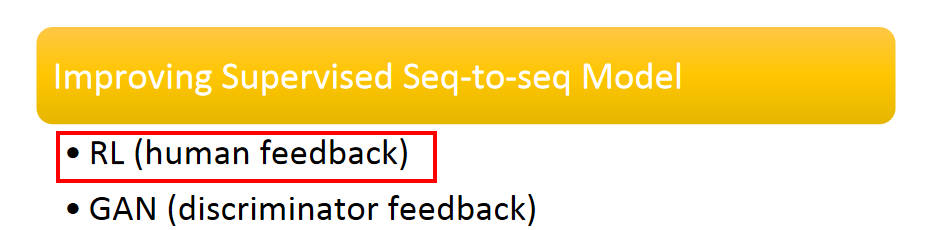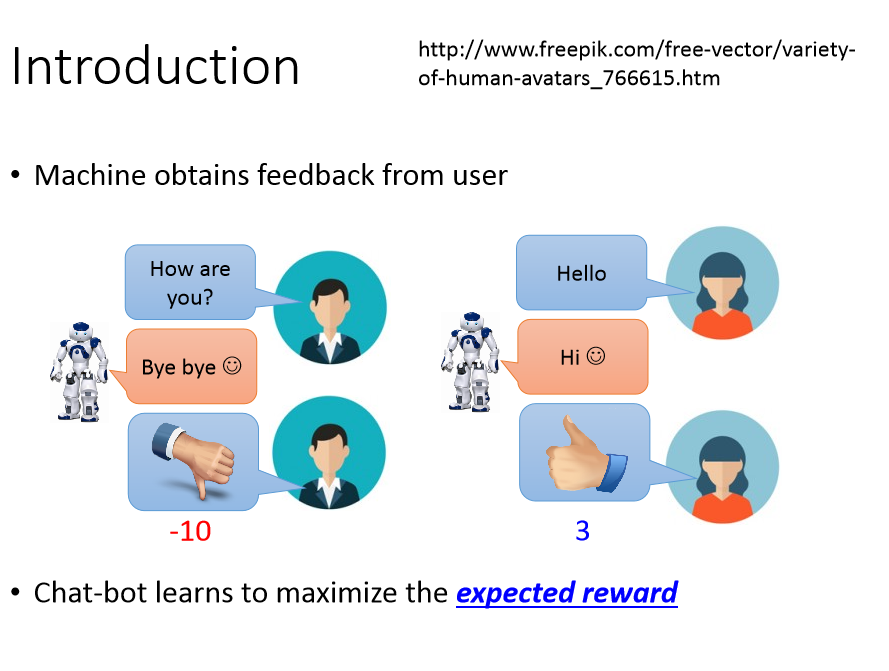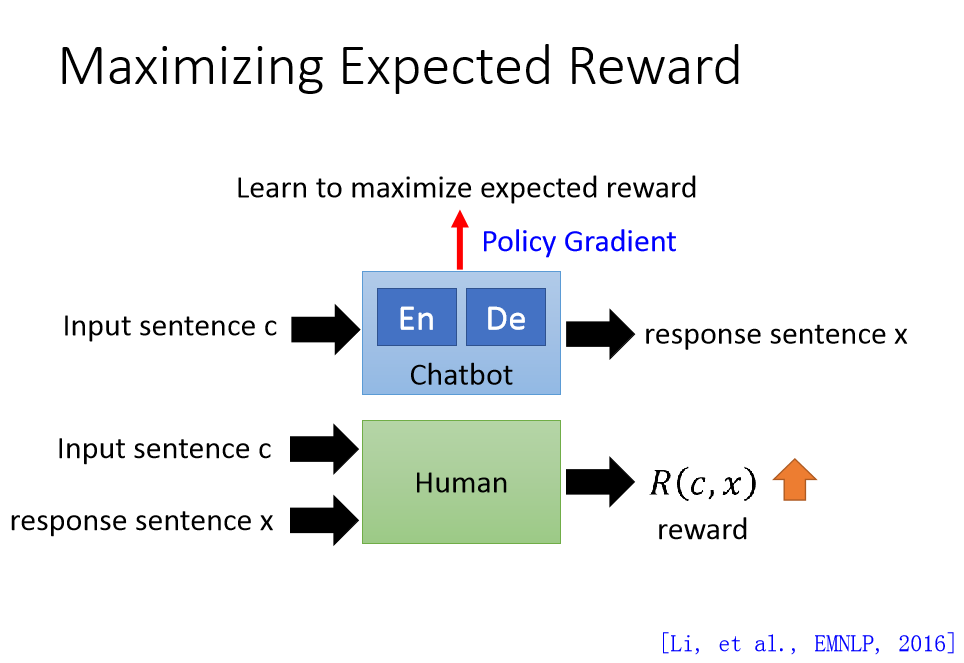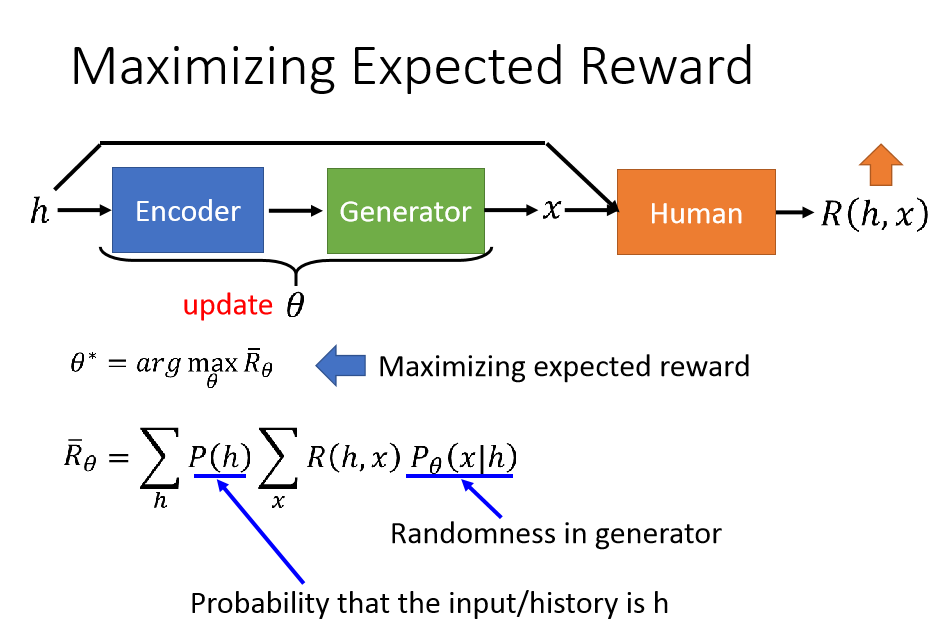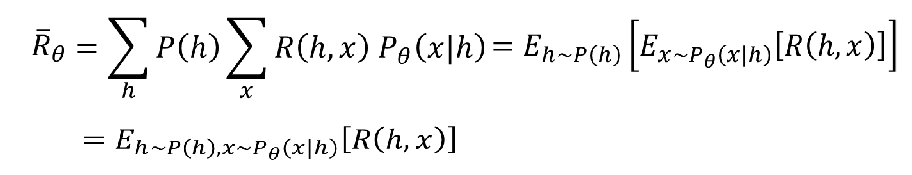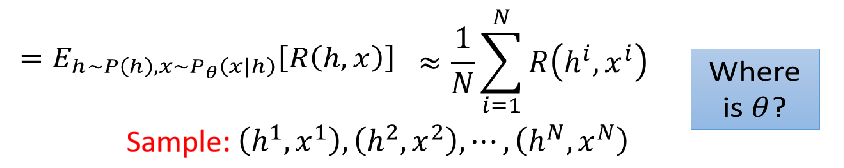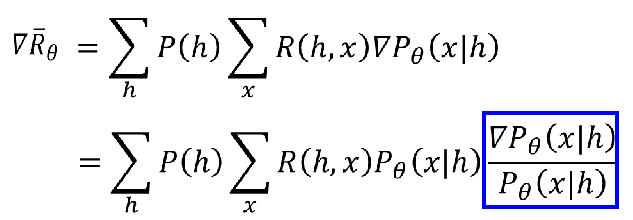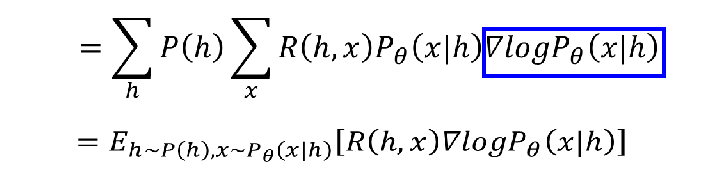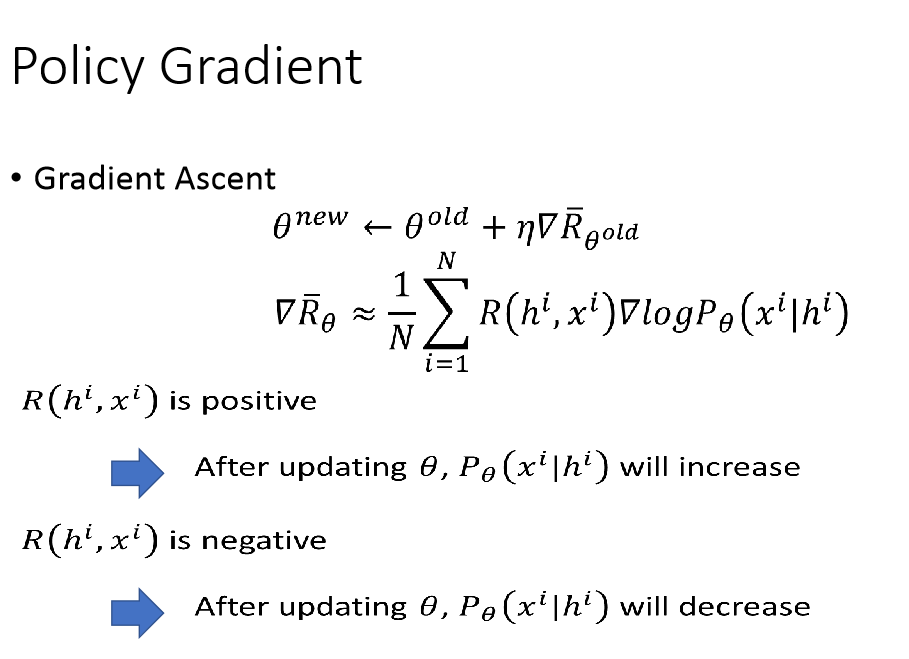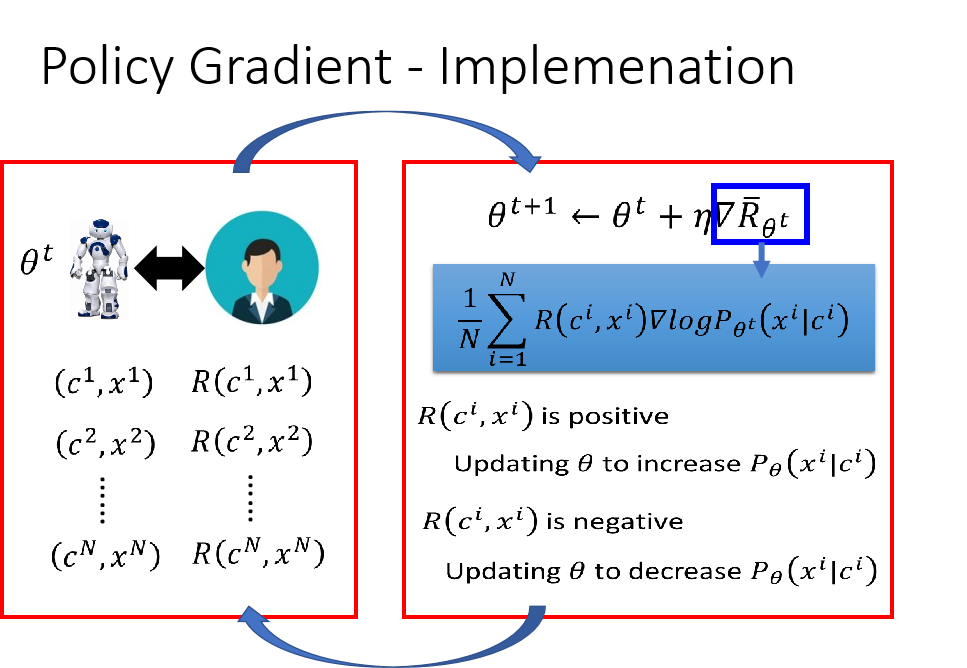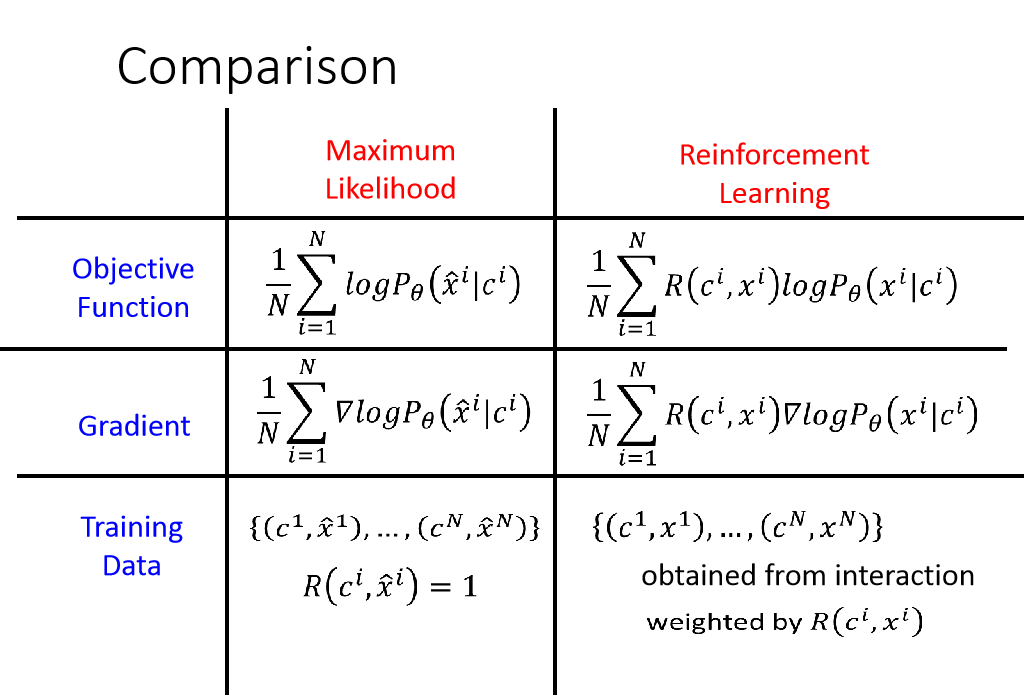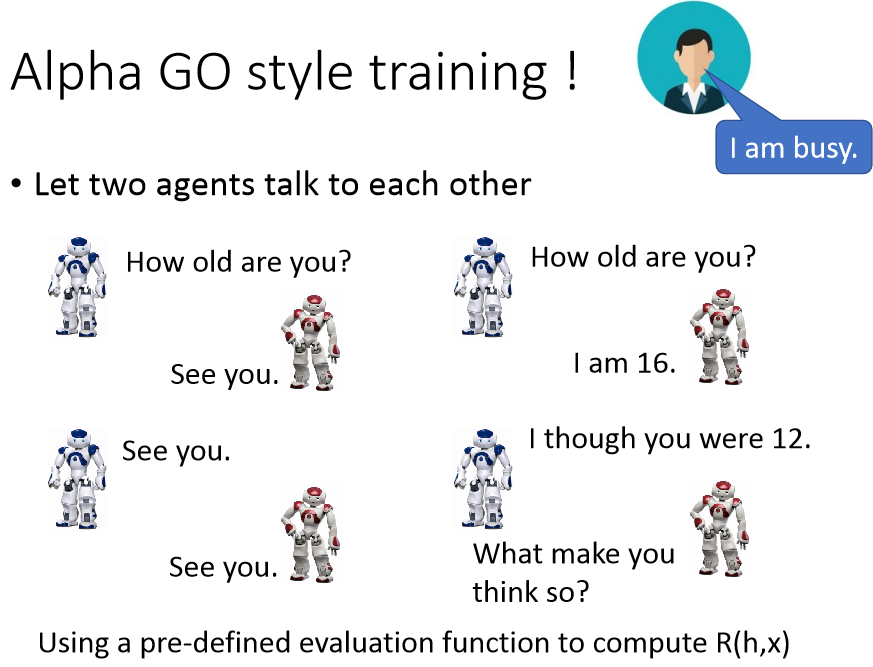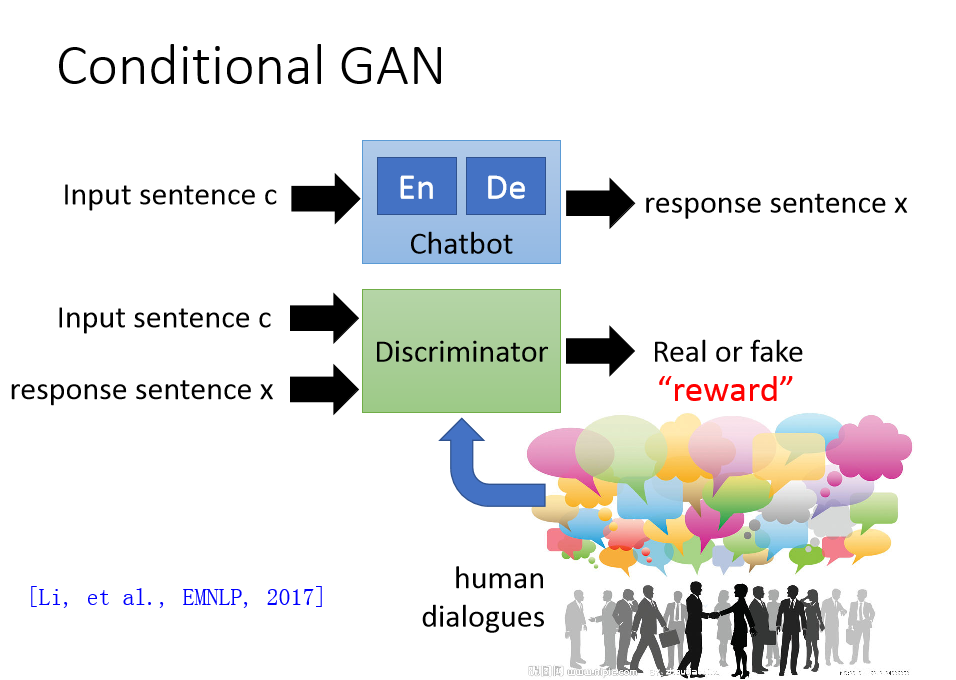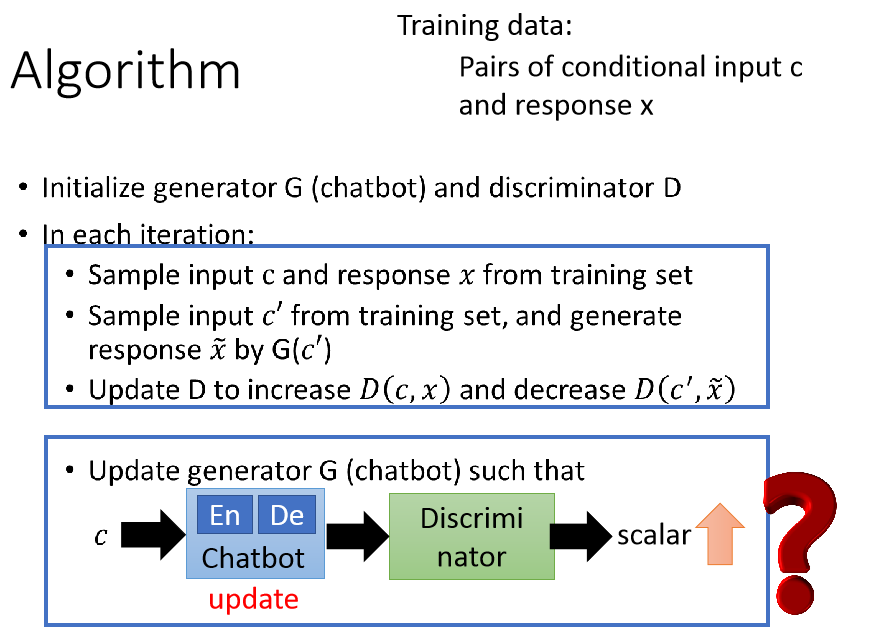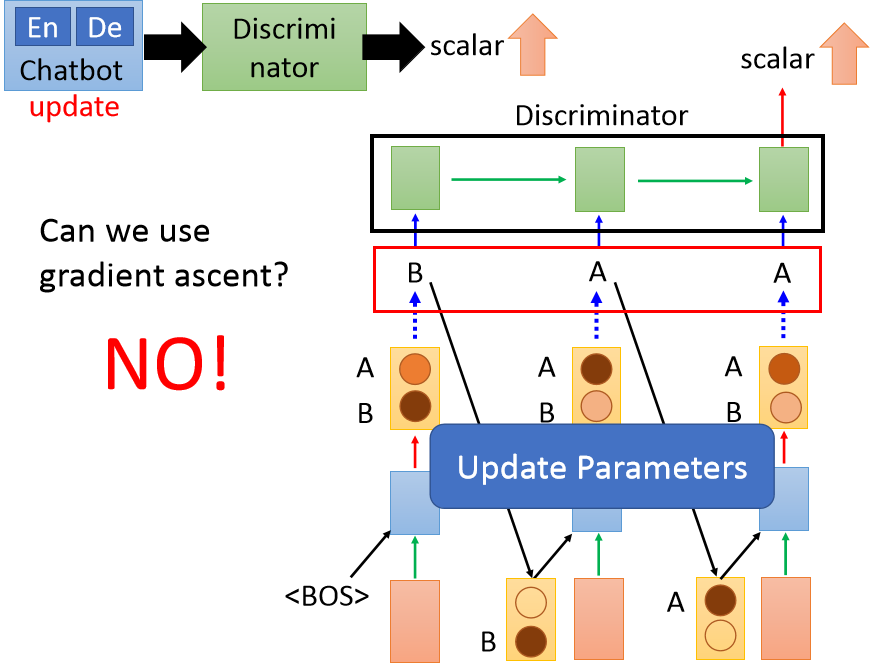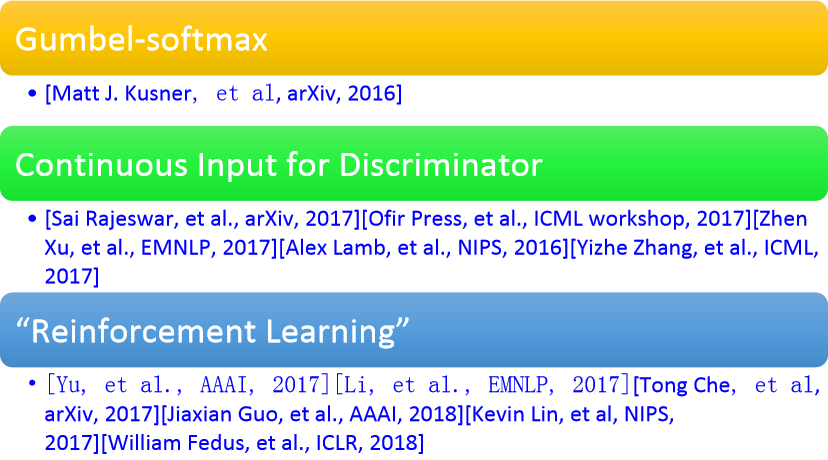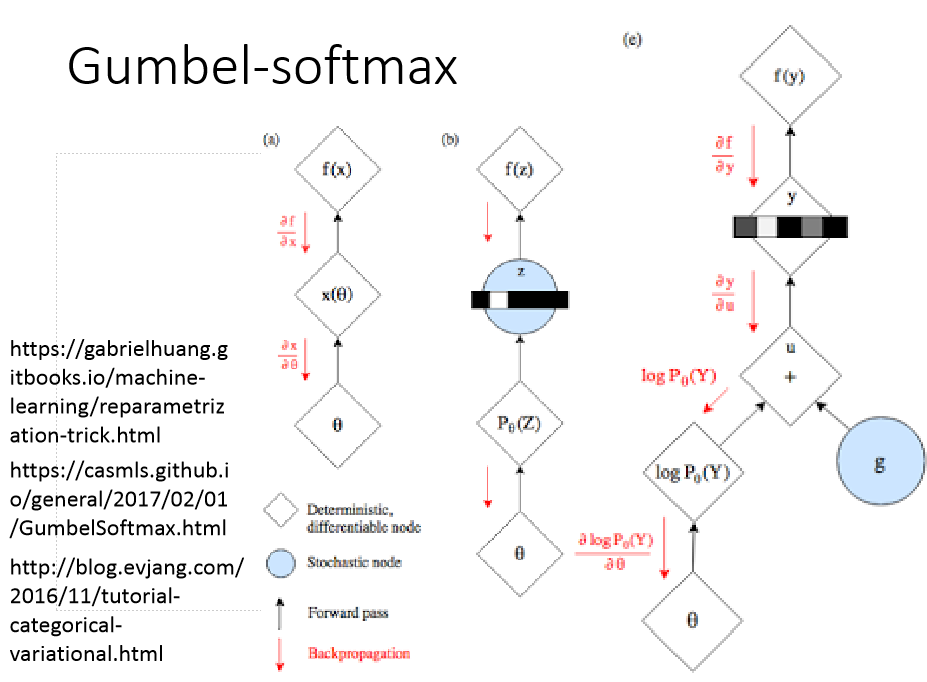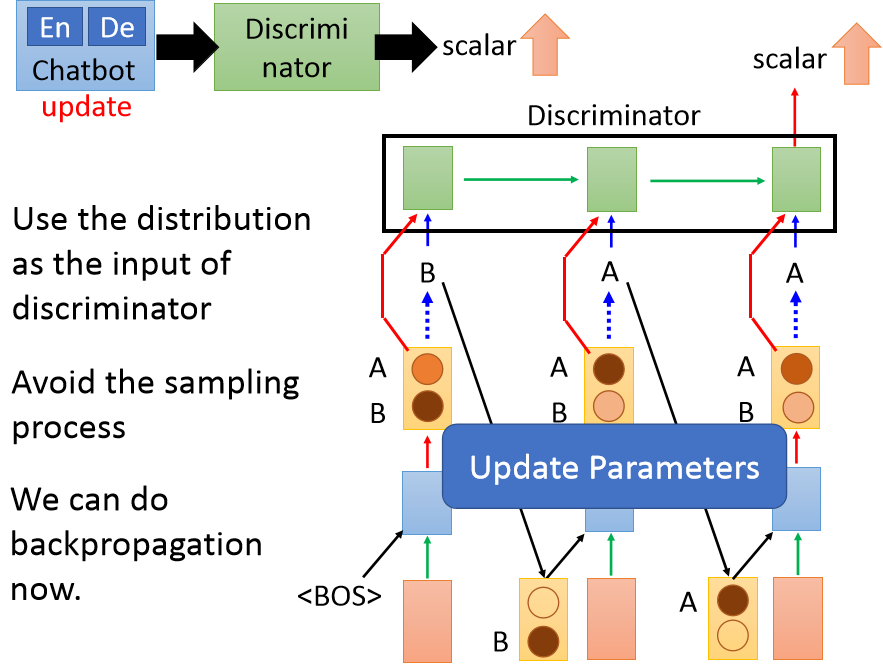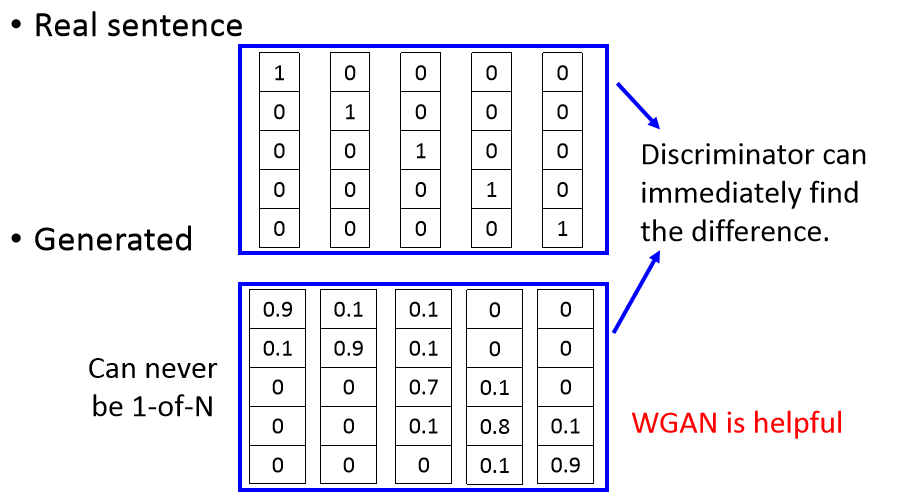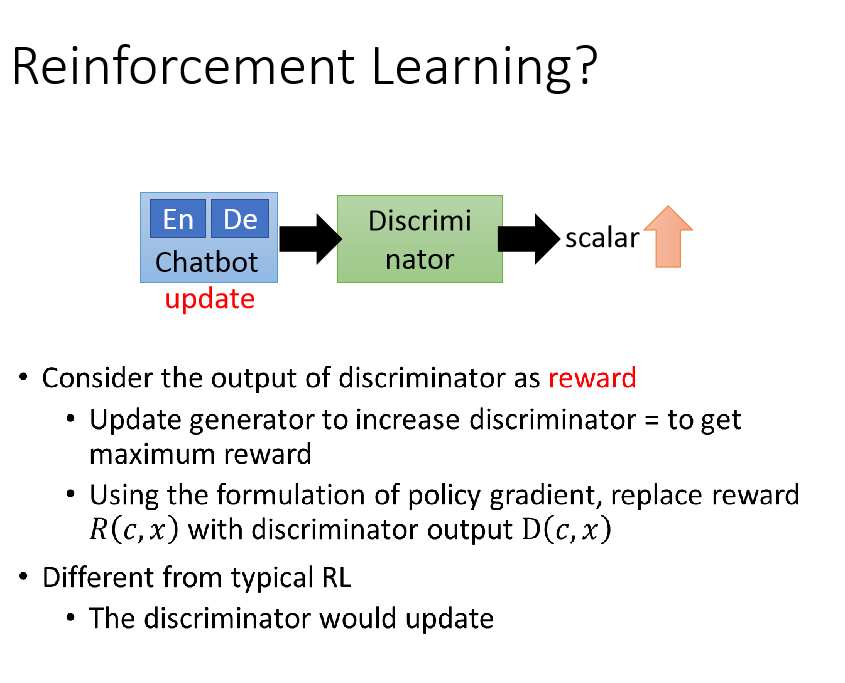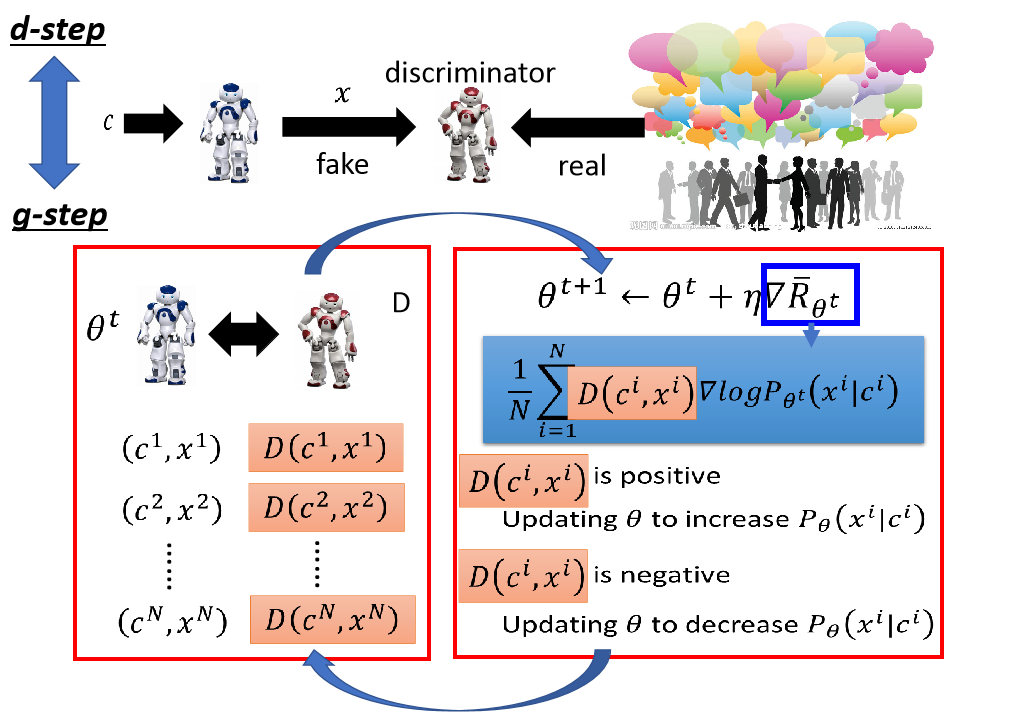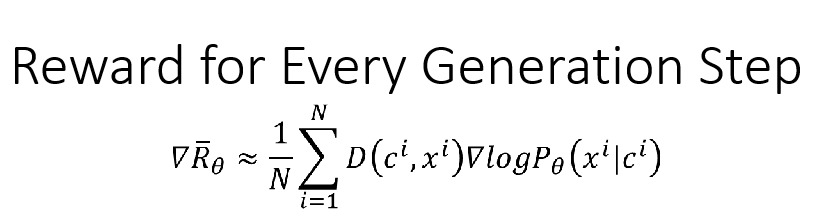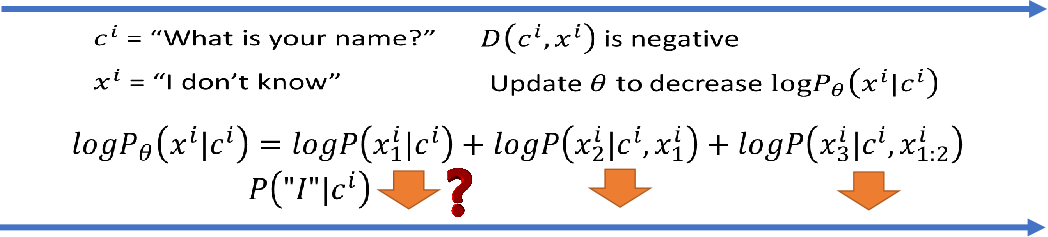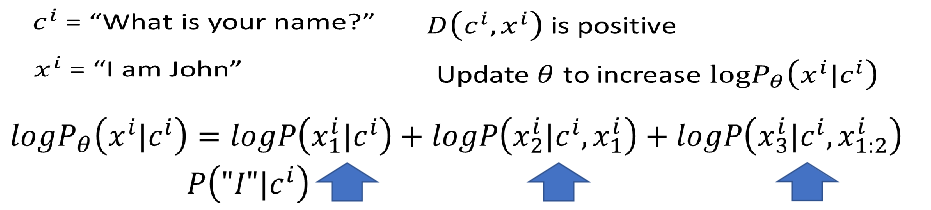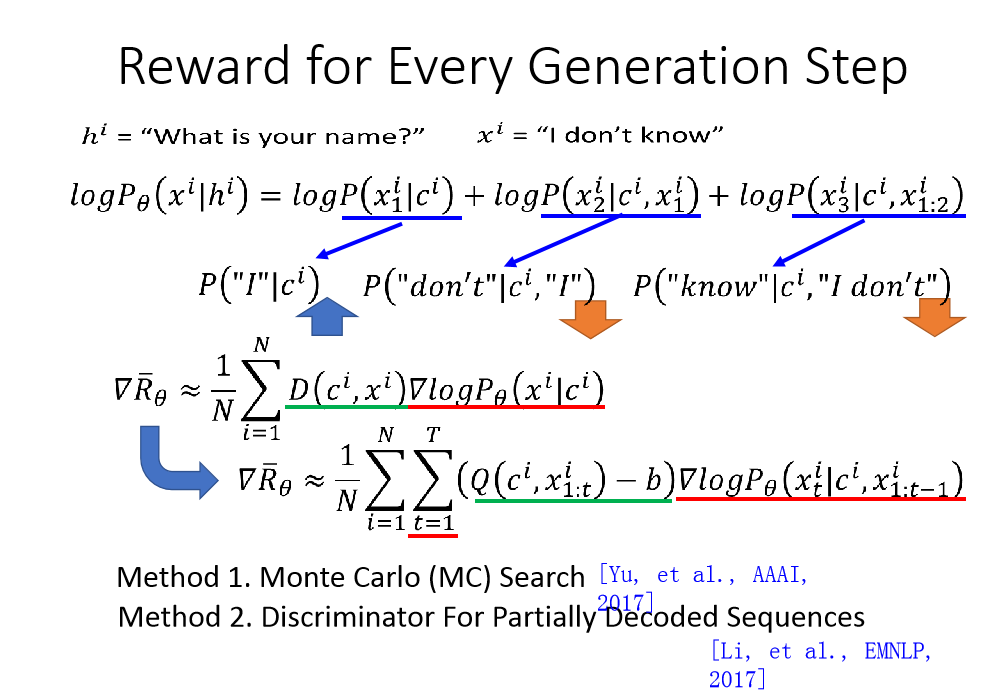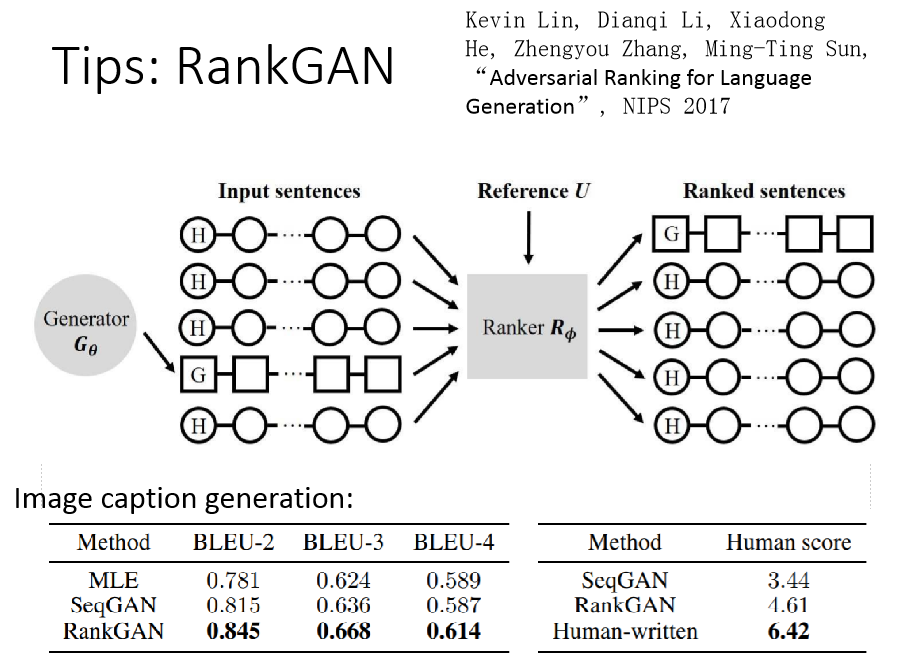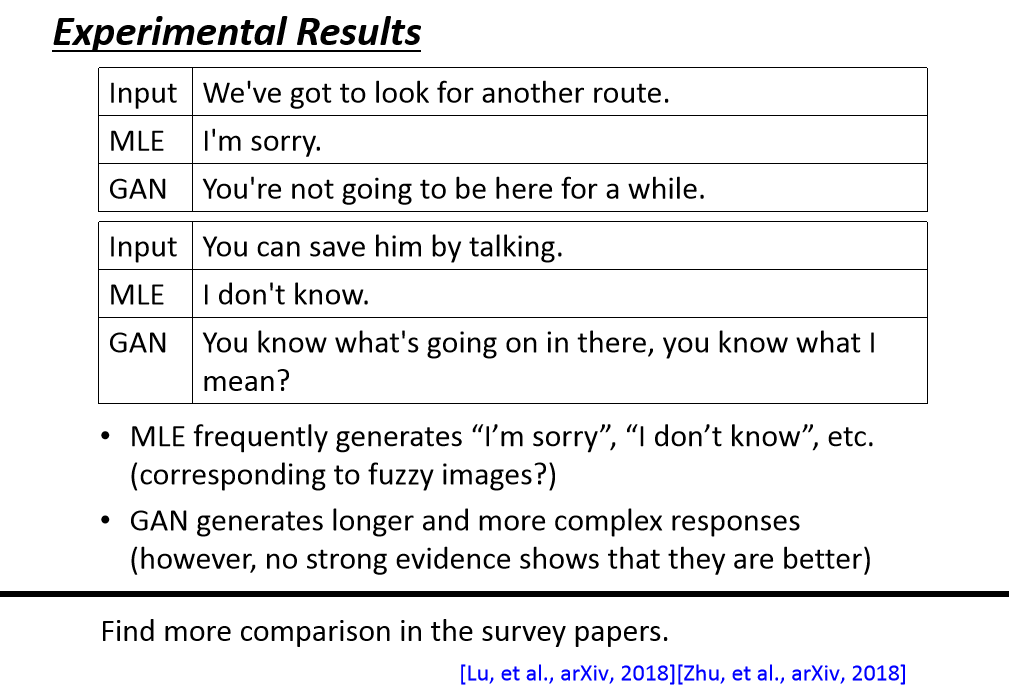2019-09-09 21:27:29 ACBC12345 阅读数 531
• ###### 深度学习入门及如何转型AI领域

深度学习入门转型视频教程，该课程主要告诉开发者如何快速入门深度学习。讲师是在机器学习，深度学习神经网络领域多年开发研究经验，精通算法原理与编程实践。曾完成过多项图像识别，目标识别，语音识别的实际项目，经验丰富。关注深度学习领域各种开源项目，如TensorFlow，Caffe，Torch等。喜欢理论与实践相结合的教学风格，课程编排由浅入深，体系清晰完整。

2772 人正在学习 去看看 CSDN讲师

WaveNet: 原始音频生成模型Tacotron:端到端的语音合成Deep Voice 1: 实时神经文本语音转换Deep Voice 2: 多说话人神经文本语音转换Deep Voice 3: 带有卷积序列学习的尺度文本语音转换Parallel WaveNet: 快速高保真语音合成利用小样本的神经网络语音克隆VoiceLoop: 通过语音循环进行语音拟合与合成利用梅尔图谱预测上的条件WaveNet进行自然TTS合成WaveNet: 原始音频生成模型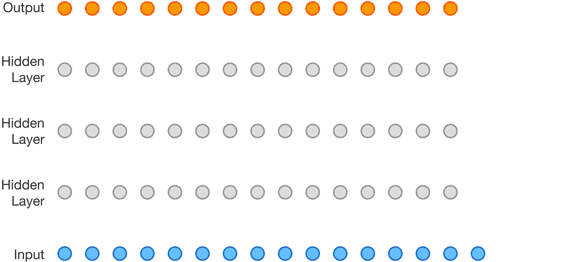WaveNET是基于PixelCNN的音频生成模型，它能够产生类似于人类发出的声音。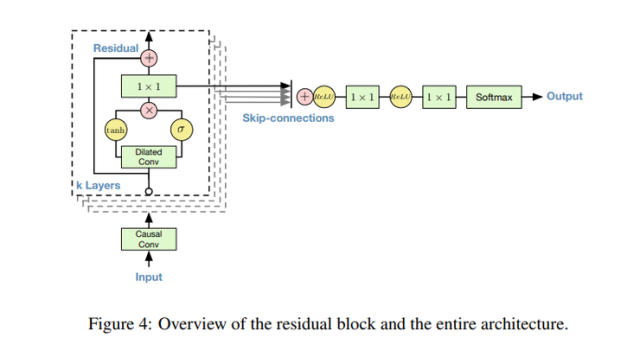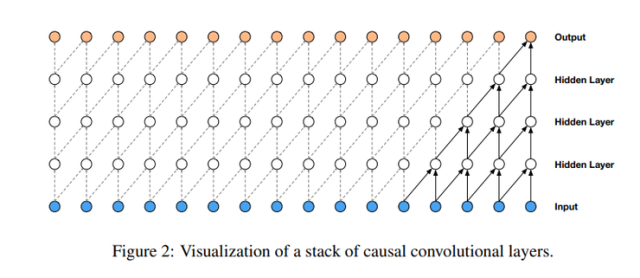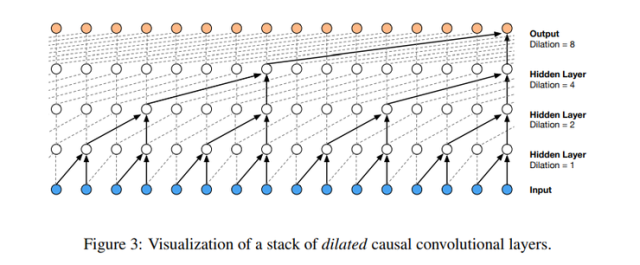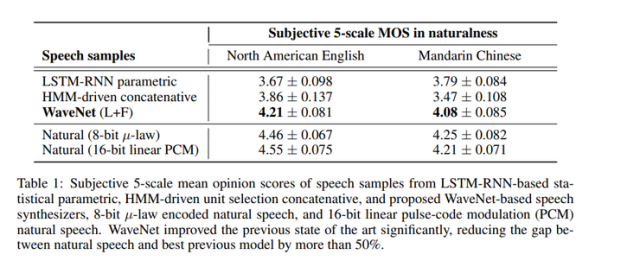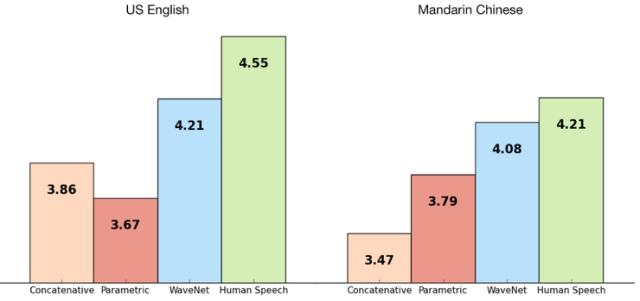Tacotron:端到端的语音合成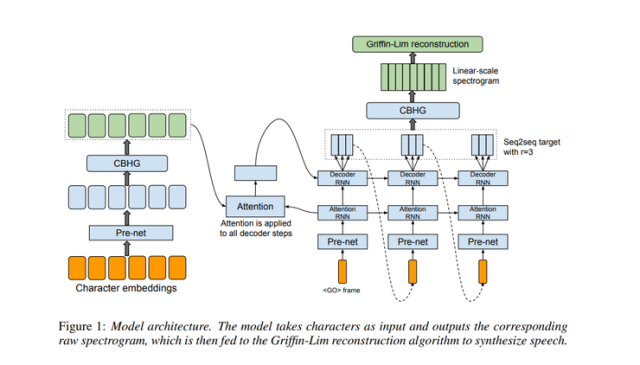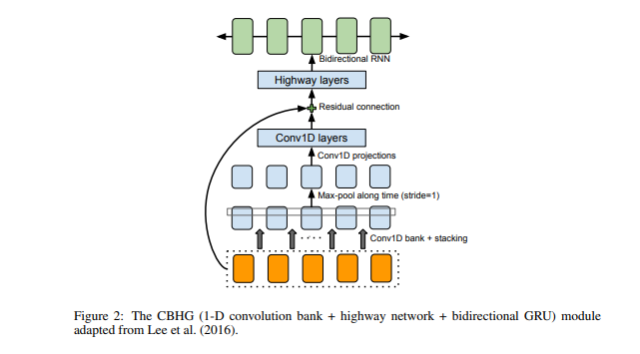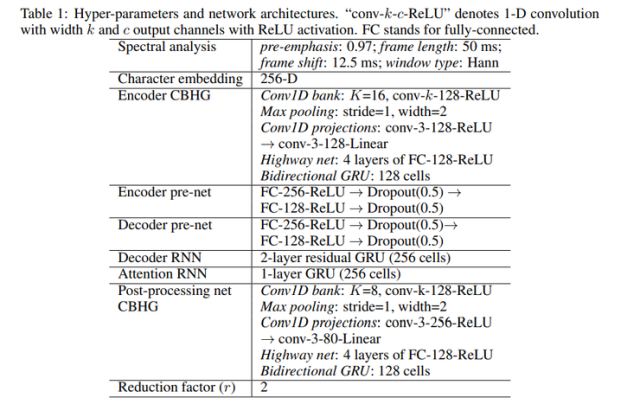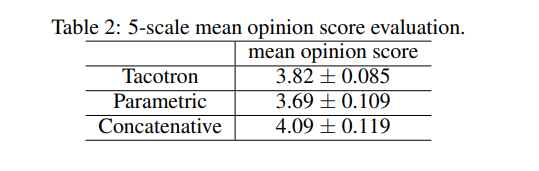Deep Voice 1: 实时神经文本到语音合成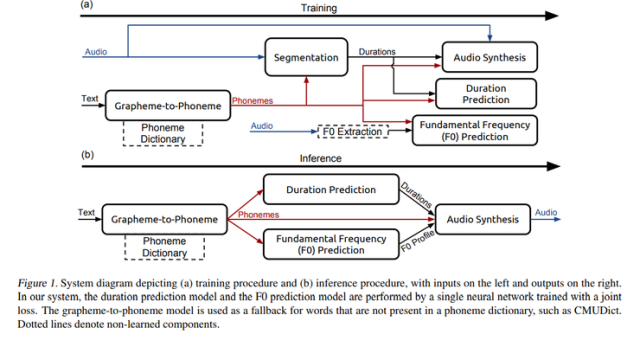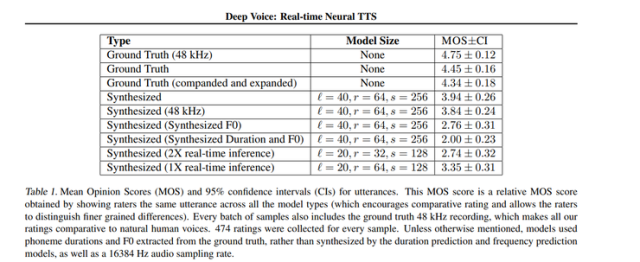Deep Voice 2: 多说话人神经文本语音转换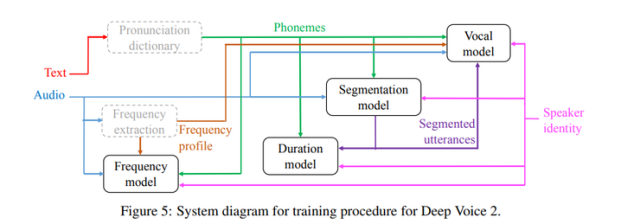Deep Voice 2和Deep Voice 1之间的主要区别在于音素持续时间模型和频率模型的分离。 Deep Voice 1有一个用于联合预测音素持续时间和频率曲线的单一模型; 而在Deep Voice 2中，则先预测音素持续时间，然后将它们用作频率模型的输入。

Deep Voice 2中的分割模型使用一种卷积递归结构（采用连接时间分类(CTC)损失函数）对音素对进行分类。Deep Voice 2的主要修改是在卷积层中添加了大量的归一化和残余连接。它的发声模型是基于WaveNet架构的。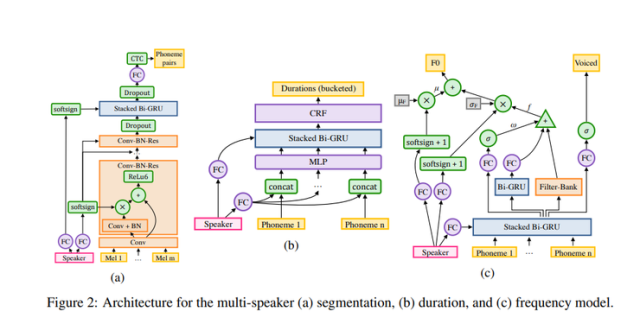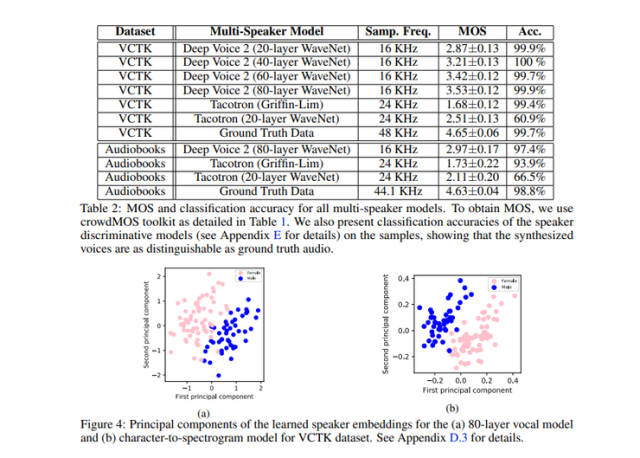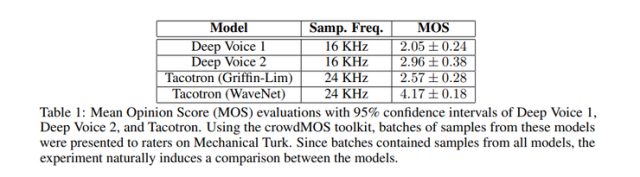Deep Voice 3: 利用卷积序列学习将文本转换为语音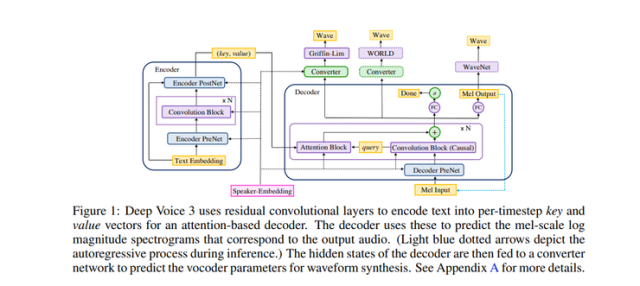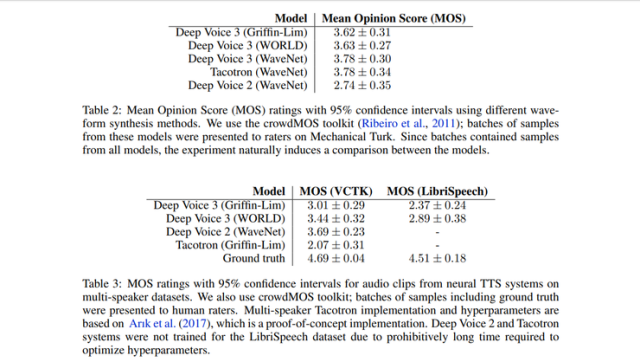Parallel WaveNet: 快速高保真语音合成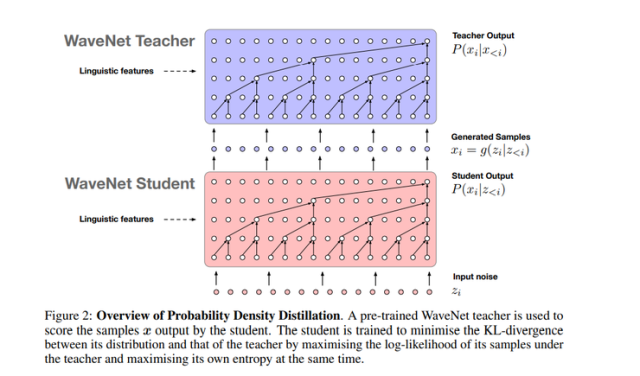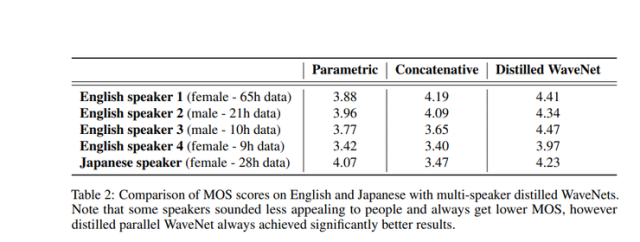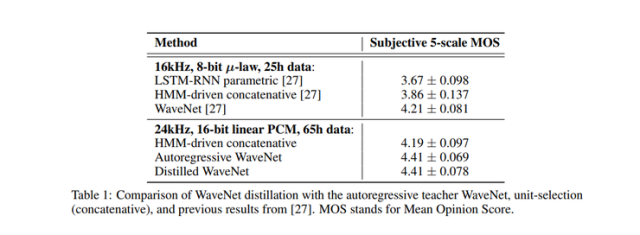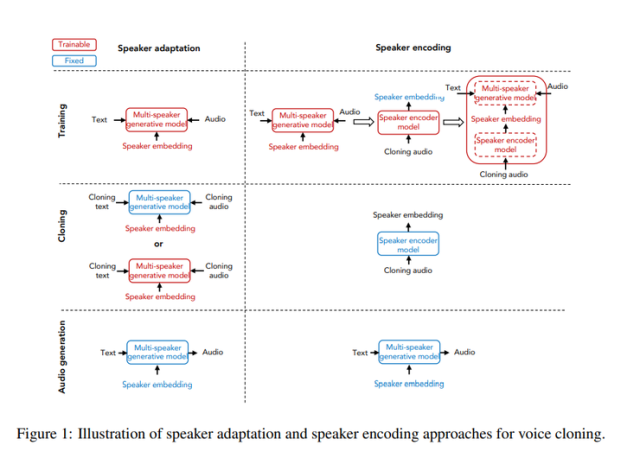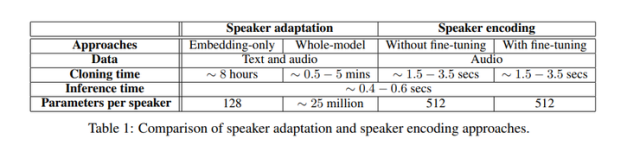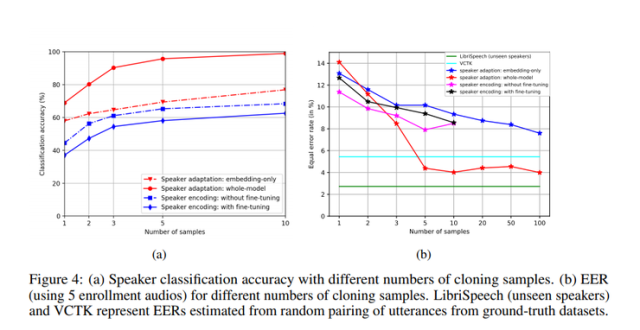VoiceLoop: 通过语音循环进行语音拟合与合成

VoiceLoop的灵感来源于一种称为语音循环的工作记忆模型，它能在短时间内保存语言信息。它由两部分组成，其一是一个不断被替换的语音存储（phonological store），其二是一个在语音存储中保持长期表达（longer-term representations）的预演过程。

Voiceloop将移动缓冲区视作矩阵，从而来构造语音存储。句子表示为音素列表。然后从每个音素解码一个短向量。通过对音素的编码进行加权并在每个时间点对它们求和来生成当前的上下文向量。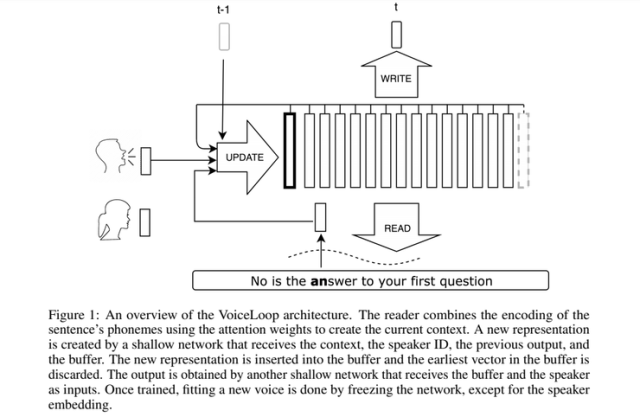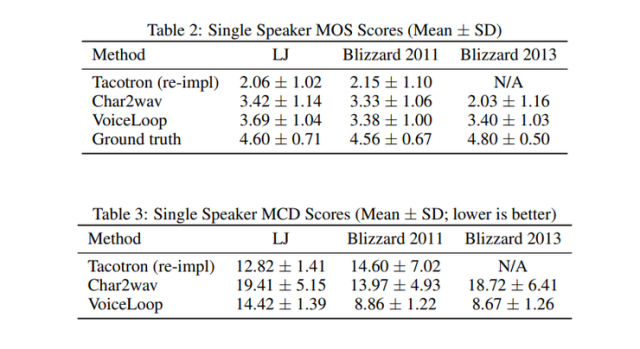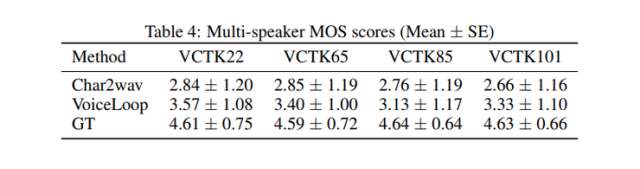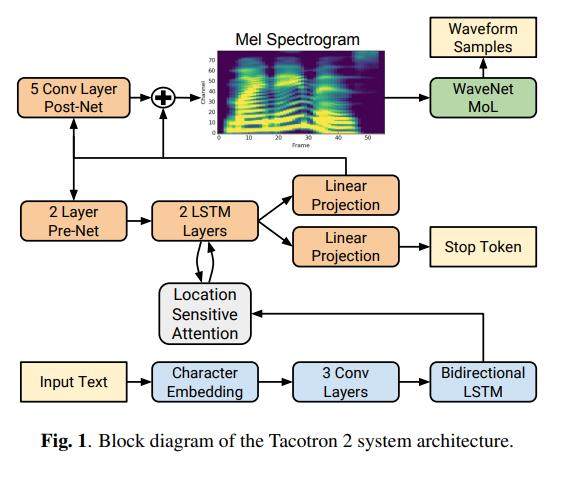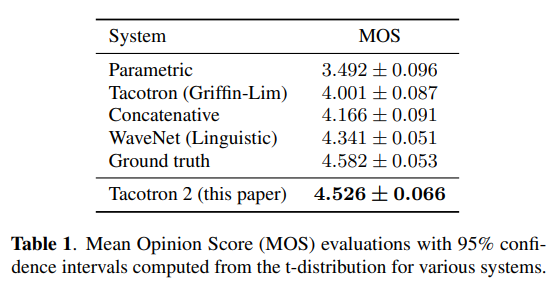https://heartbeat.fritz.ai/a-2019-guide-to-speech-synthesis-with-deep-learning-630afcafb9dd

2018-05-29 11:41:08 qq_41185868 阅读数 10413
• ###### 深度学习入门及如何转型AI领域

深度学习入门转型视频教程，该课程主要告诉开发者如何快速入门深度学习。讲师是在机器学习，深度学习神经网络领域多年开发研究经验，精通算法原理与编程实践。曾完成过多项图像识别，目标识别，语音识别的实际项目，经验丰富。关注深度学习领域各种开源项目，如TensorFlow，Caffe，Torch等。喜欢理论与实践相结合的教学风格，课程编排由浅入深，体系清晰完整。

2772 人正在学习 去看看 CSDN讲师

Python之GUI：基于Python的GUI界面设计的一套AI课程学习(机器学习、深度学习、大数据、云计算等)推荐系统(包括语音生成、识别等前沿黑科技)

## 输出结果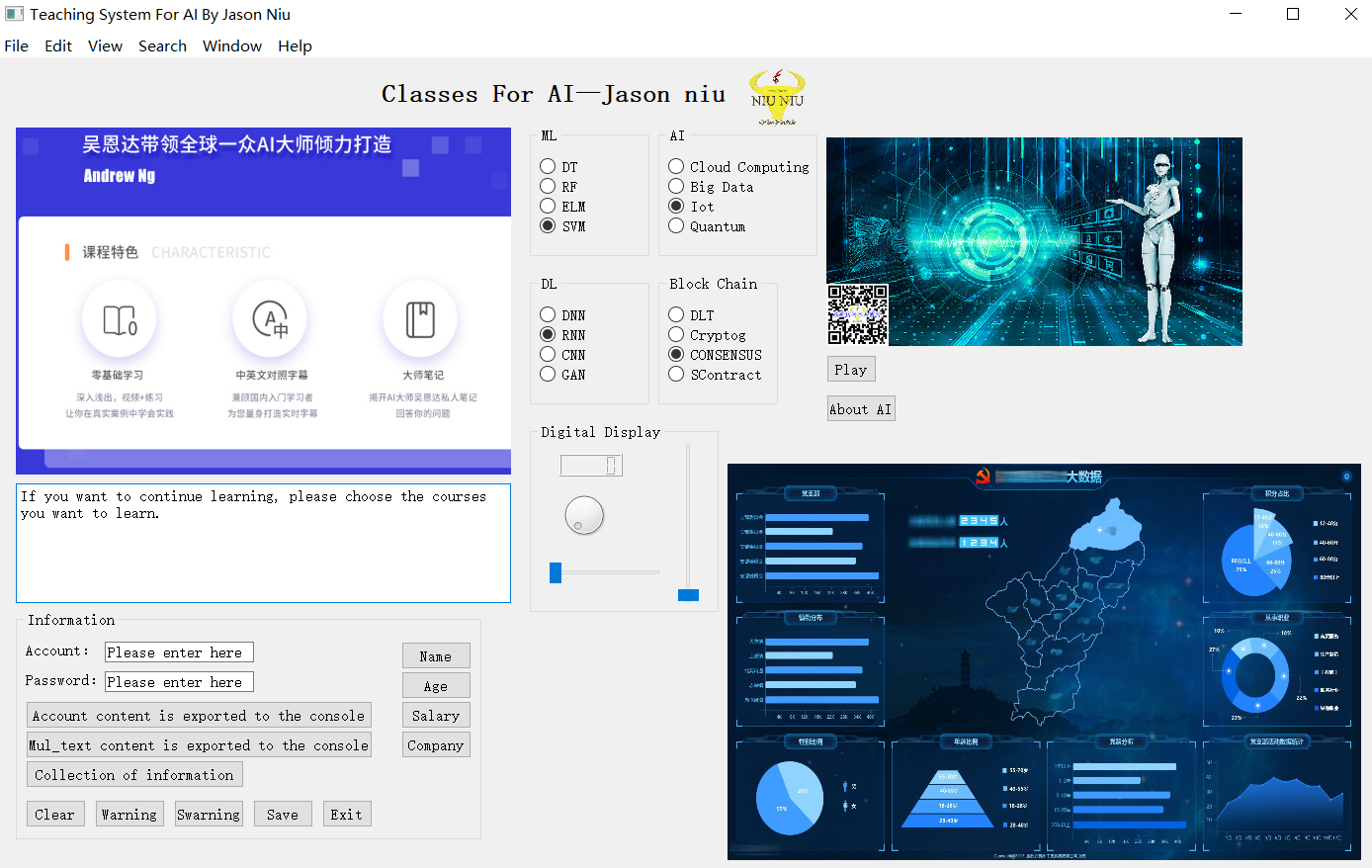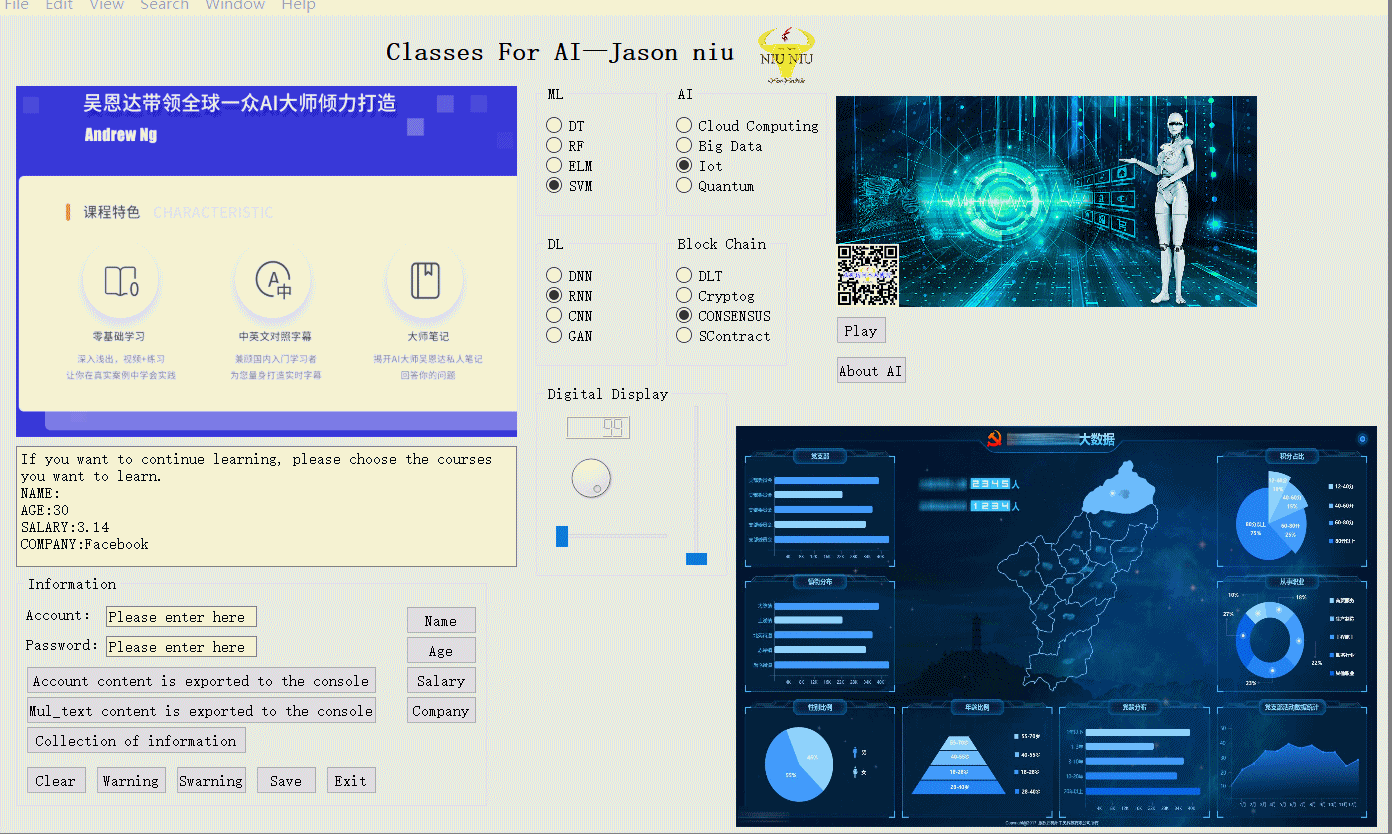## 核心代码

def niu_read_docx(filename):
doc=docx.Document(filename)
fulltext=[]
for para in doc.paragraphs:
fulltext.append(para.text)
return '\n'.join(fulltext)

class dialog(QDialog, Ui_dialog):
"""
Class documentation goes here.
"""
def __init__(self, parent=None):
"""
Constructor

@param parent reference to the parent widget
@type QWidget
"""
super(dialog, self).__init__(parent)
self.setupUi(self)

time.sleep(2) #使开机画面停留2秒

@pyqtSlot()
def on_pushButton_clicked(self):
"""
Slot documentation goes here.
"""
my_str_2=self.lineEdit_2.text()
my_str_3=self.lineEdit_3.text()
my_str_4=self.lineEdit_4.text()

print(my_str, my_str_2, my_str_3, my_str_4)
Button1_1=QMessageBox.information(self, u'提示信息框', u'输入的s所有信息已经存储到数据库中！', ) #提示对话信息框
print('OK')
self.close() #关闭当前界面

@pyqtSlot()
def on_pushButton_2_clicked(self):
"""
Slot documentation goes here.
"""
print('Cancel')

class MainWindow(QMainWindow, Ui_MainWindow):
"""
Class documentation goes here.
"""
def __init__(self, parent=None):
"""
Constructor
@param parent reference to the parent widget
@type QWidget
"""
super(MainWindow, self).__init__(parent)
self.setupUi(self)
self.graphicsView.mousePressEvent=self.my_clicked

def my_clicked(self, e):
print('自定义的点击事件函数')
webbrowser.open('www.baidu.com') #默认浏览器打开指定网址

@pyqtSlot()
def on_pushButton_clicked(self):
"""
Slot documentation goes here.
"""
print(self.textBrowser.toPlainText())

@pyqtSlot()
def on_pushButton_2_clicked(self):
"""
Slot documentation goes here.
这是测试按钮的槽函数
"""
self.lineEdit.setText( "")  #清除单行文本框内容
self.lineEdit_2.setText( "")
self.textBrowser.setText( "") #清除多行文本框内容
print("清除登陆账号信息！")

@pyqtSlot()
def on_pushButton_3_clicked(self):
"""
Slot documentation goes here.
"""
my_str=self.lineEdit.text()+":"+self.lineEdit_2.text() #获取单行文本框的内容
self.textBrowser.append(my_str) #向多行文本添加内容
print(my_str)

@pyqtSlot()
def on_pushButton_4_clicked(self):
"""
Slot documentation goes here.
该按钮命令调用的预定义的函数，退出的命令
"""

@pyqtSlot()
def on_pushButton_5_clicked(self):
"""
Slot documentation goes here.
"""
Button5=QMessageBox.question(self, u'提示信息框', u'是否全部保存到数据库中？')
if Button5==0:
print('全部保存中……')
else:
print('没有保存')

@pyqtSlot()
def on_pushButton_6_clicked(self):
"""
Slot documentation goes here.
"""
Button6=QMessageBox.warning(self, u'提警告信息框', u'没有警告信息，请继续输入！')

@pyqtSlot()
def on_pushButton_7_clicked(self):
"""
Slot documentation goes here.
"""
Button7=QMessageBox.critical(self, u'严重警告！', u'没有严重警告信息，请继续输入！')

@pyqtSlot()
def on_pushButton_9_clicked(self):
"""
Slot documentation goes here.
"""
self.graphicsView.setStyleSheet("border-image: url(:/im/image/AI (4).jpg);")

#    @pyqtSlot(QUrl)
def on_textBrowser_anchorClicked(self, p0):
"""
Slot documentation goes here.

@param p0 DESCRIPTION
@type QUrl
"""

@pyqtSlot()
"""
Slot documentation goes here.
"""
self.label_4.setStyleSheet("border-image: url(:/im/image/AI (4).jpg);")

2017-09-12 13:34:52 qq_36852006 阅读数 784
• ###### 深度学习入门及如何转型AI领域

深度学习入门转型视频教程，该课程主要告诉开发者如何快速入门深度学习。讲师是在机器学习，深度学习神经网络领域多年开发研究经验，精通算法原理与编程实践。曾完成过多项图像识别，目标识别，语音识别的实际项目，经验丰富。关注深度学习领域各种开源项目，如TensorFlow，Caffe，Torch等。喜欢理论与实践相结合的教学风格，课程编排由浅入深，体系清晰完整。

2772 人正在学习 去看看 CSDN讲师Siri 的 TTS 系统的目标是训练一个基于深度学习的统一模型，该模型能自动准确地预测数据库中单元的目标成本和拼接成本。因此该方法使用深度混合密度模型来预测特征值的分布。这种网络结构结合了常规的深度神经网络和高斯混合模型的优势，即通过 DNN 对输入和输出之间的复杂关系进行建模，并且以概率分布作为输出。系统使用了基于 MDN 统一的目标和拼接模型，该模型能预测语音目标特征（谱、基频、时长）和拼接成本分布，并引导基元的搜索。对于元音，有时语音特征相对稳定，而有些时候变化又非常迅速，针对这一问题，模型需要能够根据这种变化性对参数作出调整，因此在模型中使用嵌入方差解决这一问题。系统在运行速度、内存使用上具有一定优势，使用快速预选机制、单元剪枝和计算并行化优化了它的性能，可以在移动设备上运行。

1. 预测幅值谱信息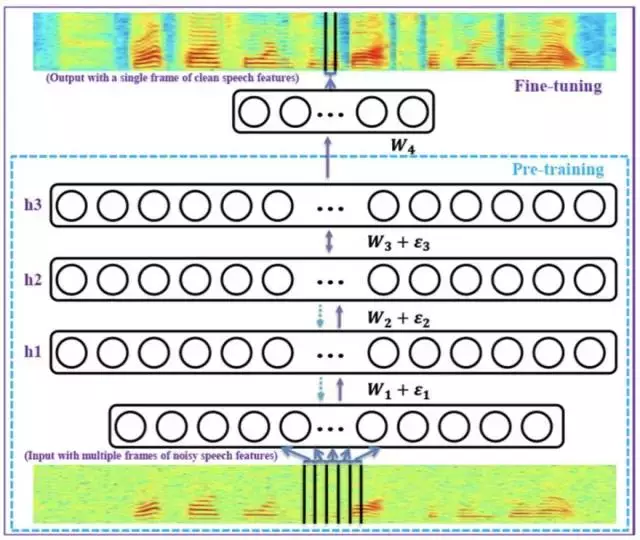2. 预测屏蔽值信息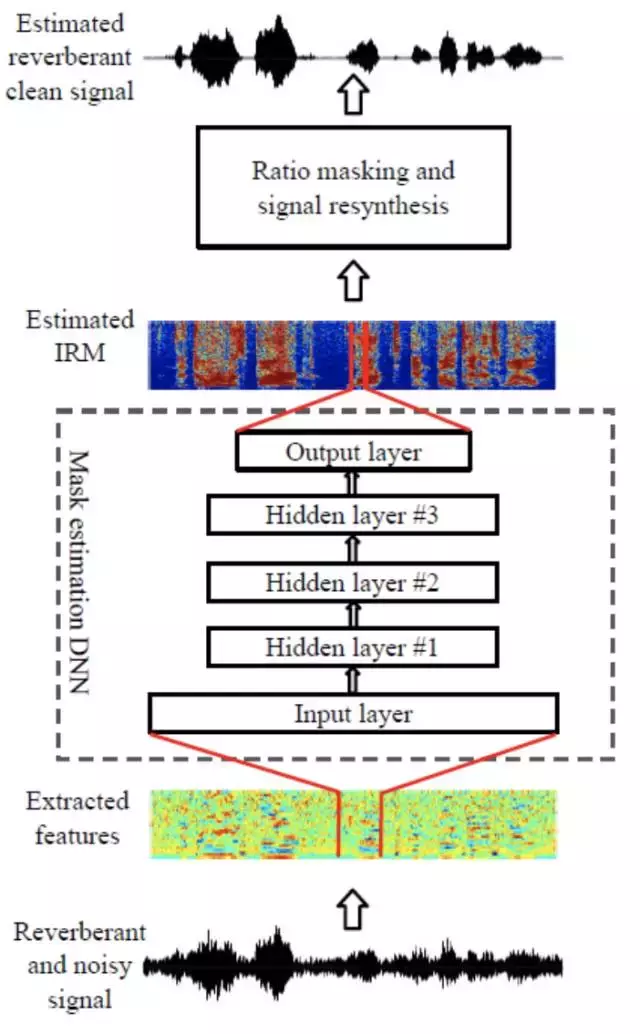3. 预测复数谱信息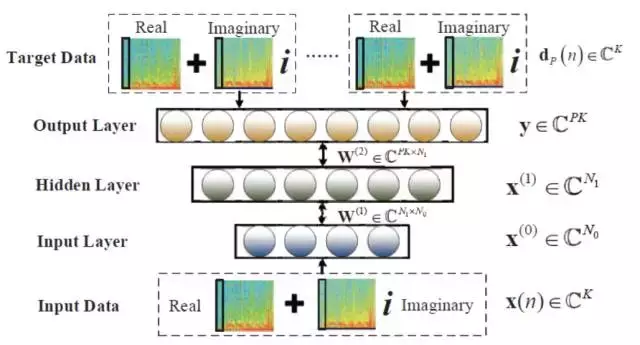4. PIT 说话人分离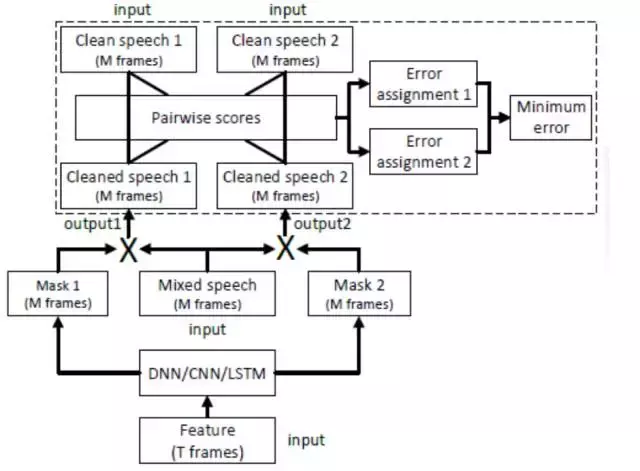5. DeepClustering 说话人分离

6. 基于对抗网络的语音增强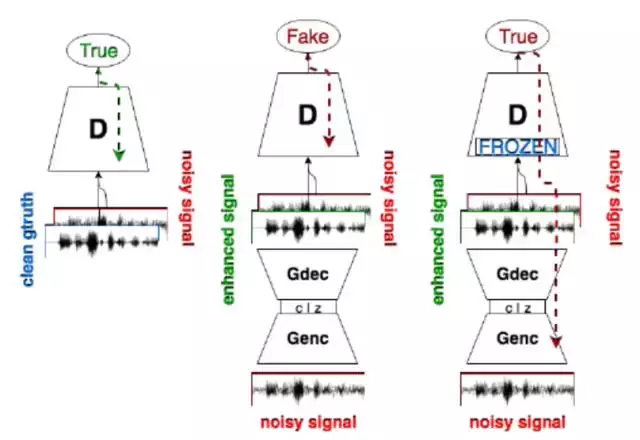2018-12-21 00:45:04 weixin_37993251 阅读数 270
• ###### 深度学习入门及如何转型AI领域

深度学习入门转型视频教程，该课程主要告诉开发者如何快速入门深度学习。讲师是在机器学习，深度学习神经网络领域多年开发研究经验，精通算法原理与编程实践。曾完成过多项图像识别，目标识别，语音识别的实际项目，经验丰富。关注深度学习领域各种开源项目，如TensorFlow，Caffe，Torch等。喜欢理论与实践相结合的教学风格，课程编排由浅入深，体系清晰完整。

2772 人正在学习 去看看 CSDN讲师

# 第7章 生成式深度学习

• 使用LSTM生成文本
• 实现DeepDream
• 实现神经风格迁移
• 变分自编码器
• 了解生成式对抗网络

## 8.1 使用LSTM生成文本

### 8.1.1 生成式循环网络简史

Schmidhuber：LSTM 1997

### 8.1.3 采样策略的重要性

# 8-1 对于不同的softmax温度，对概率分布重新加权
import numpy as np

def reweight_distribution(original_distribution, temperature = 0.5):
distribution = np.log(original_distribution) / temperature
distribution = np.exp(distribution)
return distribution / np.sum(distribution)


### 8.1.4 实现字符级的LSTM文本生成

1. 准备数据
# 8-2 下载并解析初始文本文件
import keras
import numpy as np

path = keras.utils.get_file(
'nietzsche.txt',
origin='https://s3.amazonaws.com/text-datasets/nietzsche.txt')
print('Corpus length:', len(text))


# 8-3 将字符序列向量化
maxlen = 60 # 提取60个字符组成的序列

step = 3 # 每3个字符采集一个新序列

sentences = [] # 保存所提取的序列

next_chars = [] # 保存目标

for i in range(0, len(text) - maxlen, step):
sentences.append(text[i: i + maxlen])
next_chars.append(text[i + maxlen])

print('Number of sentences:', len(sentences))

# 将唯一字符映射在列表中
chars = sorted(list(set(text)))
print('Unique characters:', len(chars))
char_indices = dict((char, chars.index(char)) for char in chars)

# one-hot编码
print('Vectorization')
x = np.zeros((len(sentences), maxlen, len(chars)), dtype = np.bool)
y = np.zeros((len(sentences), len(chars)), dtype = np.bool)
for i, sentence in enumerate(sentences):
for t, char in enumerate(sentence):
x[i, t, char_indices[char]] = 1
y[i, char_indices[next_chars[i]]] = 1


Number of sentences: 200278
Unique characters: 58
Vectorization

1. 构建网络
# 8-4 用于预测下一个字符的单层LSTM模型
from keras import layers

model = keras.models.Sequential()


# 8-5 模型编译配置
optimizer = keras.optimizers.RMSprop(lr=0.01)
model.compile(loss='categorical_crossentropy', optimizer=optimizer)

1. 训练语言模型并从中采样
• 给定已经生成的文本，从模型中得到下一个字符的概率分布
• 根据某个温度对分布进行重新加权
• 根据重新加权后的分布对下一个字符进行随机采样
• 将新字符添加到文本末尾

# 8-7 文本生成循环
import random
import sys

for epoch in range(1, 60):
print('epoch', epoch)
# Fit the model for 1 epoch on the available training data
model.fit(x, y,
batch_size=128,
epochs=1)

# Select a text seed at random
start_index = random.randint(0, len(text) - maxlen - 1)
generated_text = text[start_index: start_index + maxlen]
print('--- Generating with seed: "' + generated_text + '"')

for temperature in [0.2, 0.5, 1.0, 1.2]:
print('------ temperature:', temperature)
sys.stdout.write(generated_text)

# We generate 400 characters
for i in range(400):
sampled = np.zeros((1, maxlen, len(chars)))
for t, char in enumerate(generated_text):
sampled[0, t, char_indices[char]] = 1.

preds = model.predict(sampled, verbose=0)
next_index = sample(preds, temperature)
next_char = chars[next_index]

generated_text += next_char
generated_text = generated_text[1:]

sys.stdout.write(next_char)
sys.stdout.flush()
print()


## 8.2 DeepDream

### 8.2.1 用keras实现DeepDream

Keras中有许多预训练的卷积神经网络。这里使用Keras内置的Incetion V3模型。

# 8-8 加载预训练的InceptionV3模型
from keras.applications import inception_v3
from keras import backend as K

# We will not be training our model,
# so we use this command to disable all training-specific operations
K.set_learning_phase(0)

# Build the InceptionV3 network.
# The model will be loaded with pre-trained ImageNet weights.
model = inception_v3.InceptionV3(weights='imagenet',
include_top=False)


# 8-9 设置DeepDream配置
# Dict mapping layer names to a coefficient
# quantifying how much the layer's activation
# will contribute to the loss we will seek to maximize.
# Note that these are layer names as they appear
# in the built-in InceptionV3 application.
# You can list all layer names using model.summary().
layer_contributions = {
'mixed2': 0.2,
'mixed3': 3.,
'mixed4': 2.,
'mixed5': 1.5,
}


# 8-10 定义需要最大化的损失
# Get the symbolic outputs of each "key" layer (we gave them unique names).
layer_dict = dict([(layer.name, layer) for layer in model.layers])

# Define the loss.
loss = K.variable(0.)
for layer_name in layer_contributions:
# Add the L2 norm of the features of a layer to the loss.
coeff = layer_contributions[layer_name]
activation = layer_dict[layer_name].output

# We avoid border artifacts by only involving non-border pixels in the loss.
scaling = K.prod(K.cast(K.shape(activation), 'float32'))
loss += coeff * K.sum(K.square(activation[:, 2: -2, 2: -2, :])) / scaling


# 8-11 梯度上升过程
# This holds our generated image
dream = model.input

# Compute the gradients of the dream with regard to the loss.

# Set up function to retrieve the value
# of the loss and gradients given an input image.

loss_value = outs

for i in range(iterations):
if max_loss is not None and loss_value > max_loss:
break
print('...Loss value at', i, ':', loss_value)
return x


# 8-12 在多个连续尺度上运行梯度上升
import numpy as np

# Playing with these hyperparameters will also allow you to achieve new effects

step = 0.01  # Gradient ascent step size
num_octave = 3  # Number of scales at which to run gradient ascent
octave_scale = 1.4  # Size ratio between scales
iterations = 20  # Number of ascent steps per scale

# If our loss gets larger than 10,
# we will interrupt the gradient ascent process, to avoid ugly artifacts
max_loss = 10.

# Fill this to the path to the image you want to use

# Load the image into a Numpy array
img = preprocess_image(base_image_path)

# We prepare a list of shape tuples
# defining the different scales at which we will run gradient ascent
original_shape = img.shape[1:3]
successive_shapes = [original_shape]
for i in range(1, num_octave):
shape = tuple([int(dim / (octave_scale ** i)) for dim in original_shape])
successive_shapes.append(shape)

# Reverse list of shapes, so that they are in increasing order
successive_shapes = successive_shapes[::-1]

# Resize the Numpy array of the image to our smallest scale
original_img = np.copy(img)
shrunk_original_img = resize_img(img, successive_shapes)

for shape in successive_shapes:
print('Processing image shape', shape)
img = resize_img(img, shape)
iterations=iterations,
step=step,
max_loss=max_loss)
upscaled_shrunk_original_img = resize_img(shrunk_original_img, shape)
same_size_original = resize_img(original_img, shape)
lost_detail = same_size_original - upscaled_shrunk_original_img

img += lost_detail
shrunk_original_img = resize_img(original_img, shape)
save_img(img, fname='dream_at_scale_' + str(shape) + '.png')

save_img(img, fname='final_dream.png')


# 8-13 辅助函数
import scipy
from keras.preprocessing import image

def resize_img(img, size):
img = np.copy(img)
factors = (1,
float(size) / img.shape,
float(size) / img.shape,
1)
return scipy.ndimage.zoom(img, factors, order=1)

def save_img(img, fname):
pil_img = deprocess_image(np.copy(img))
scipy.misc.imsave(fname, pil_img)

def preprocess_image(image_path):
# Util function to open, resize and format pictures
# into appropriate tensors.
img = image.img_to_array(img)
img = np.expand_dims(img, axis=0)
img = inception_v3.preprocess_input(img)
return img

def deprocess_image(x):
# Util function to convert a tensor into a valid image.
if K.image_data_format() == 'channels_first':
x = x.reshape((3, x.shape, x.shape))
x = x.transpose((1, 2, 0))
else:
x = x.reshape((x.shape, x.shape, 3))
x /= 2.
x += 0.5
x *= 255.
x = np.clip(x, 0, 255).astype('uint8')
return x

from matplotlib import pyplot as plt

plt.imshow(deprocess_image(np.copy(img)))
plt.show()


## 8.3 神经风格迁移

loss = distance(style(reference_image) - style(generated_image)) + distance(content(original_image) - content(generated_image))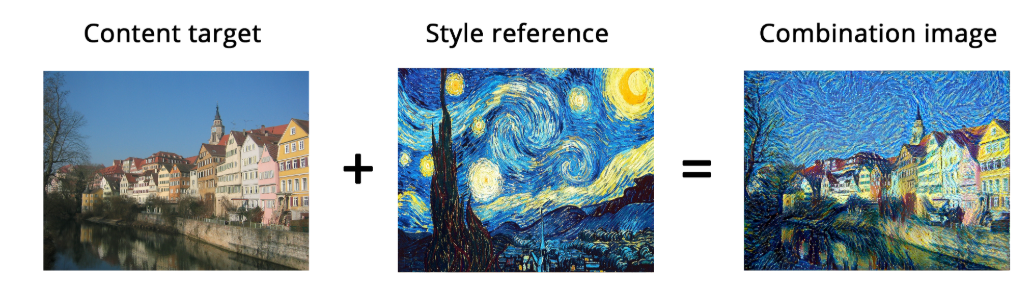### 8.3.2 风格损失

Gram matrix——特征图的内积

### 8.3.3 用Keras实现神经风格迁移

VGG19

# 8-14 定义初始变量

# This is the path to the image you want to transform.
# This is the path to the style image.

# Dimensions of the generated picture.
img_height = 400
img_width = int(width * img_height / height)


# 8-15 辅助函数
import numpy as np
from keras.applications import vgg19

def preprocess_image(image_path):
img = img_to_array(img)
img = np.expand_dims(img, axis=0)
img = vgg19.preprocess_input(img)
return img

def deprocess_image(x):
# Remove zero-center by mean pixel
x[:, :, 0] += 103.939
x[:, :, 1] += 116.779
x[:, :, 2] += 123.68
# 'BGR'->'RGB'
x = x[:, :, ::-1]
x = np.clip(x, 0, 255).astype('uint8')
return x


# 8-16 加载预训练的VGG19网络，应用于三张图片
from keras import backend as K

target_image = K.constant(preprocess_image(target_image_path))
style_reference_image = K.constant(preprocess_image(style_reference_image_path))

# This placeholder will contain our generated image
combination_image = K.placeholder((1, img_height, img_width, 3))

# We combine the 3 images into a single batch
input_tensor = K.concatenate([target_image,
style_reference_image,
combination_image], axis=0)

# We build the VGG19 network with our batch of 3 images as input.
# The model will be loaded with pre-trained ImageNet weights.
model = vgg19.VGG19(input_tensor=input_tensor,
weights='imagenet',
include_top=False)


# 8-17 内容损失
def content_loss(base, combination):
return K.sum(K.square(combination - base))

# 8-18 风格损失
def gram_matrix(x):
features = K.batch_flatten(K.permute_dimensions(x, (2, 0, 1)))
gram = K.dot(features, K.transpose(features))
return gram

def style_loss(style, combination):
S = gram_matrix(style)
C = gram_matrix(combination)
channels = 3
size = img_height * img_width
return K.sum(K.square(S - C)) / (4. * (channels ** 2) * (size ** 2))


# 总变差损失
def total_variation_loss(x):
a = K.square(
x[:, :img_height - 1, :img_width - 1, :] - x[:, 1:, :img_width - 1, :])
b = K.square(
x[:, :img_height - 1, :img_width - 1, :] - x[:, :img_height - 1, 1:, :])
return K.sum(K.pow(a + b, 1.25))


# 8-20 定义需要最小化的最终损失
# Dict mapping layer names to activation tensors
outputs_dict = dict([(layer.name, layer.output) for layer in model.layers])
# Name of layer used for content loss
content_layer = 'block5_conv2'
# Name of layers used for style loss
style_layers = ['block1_conv1',
'block2_conv1',
'block3_conv1',
'block4_conv1',
'block5_conv1']
# Weights in the weighted average of the loss components
total_variation_weight = 1e-4
style_weight = 1.
content_weight = 0.025

# Define the loss by adding all components to a loss variable
loss = K.variable(0.)
layer_features = outputs_dict[content_layer]
target_image_features = layer_features[0, :, :, :]
combination_features = layer_features[2, :, :, :]
loss += content_weight * content_loss(target_image_features,
combination_features)
for layer_name in style_layers:
layer_features = outputs_dict[layer_name]
style_reference_features = layer_features[1, :, :, :]
combination_features = layer_features[2, :, :, :]
sl = style_loss(style_reference_features, combination_features)
loss += (style_weight / len(style_layers)) * sl
loss += total_variation_weight * total_variation_loss(combination_image)


# 8-21 设置梯度下降过程
# Get the gradients of the generated image wrt the loss

# Function to fetch the values of the current loss and the current gradients

class Evaluator(object):

def __init__(self):
self.loss_value = None

def loss(self, x):
assert self.loss_value is None
x = x.reshape((1, img_height, img_width, 3))
loss_value = outs
self.loss_value = loss_value
return self.loss_value

assert self.loss_value is not None
self.loss_value = None

evaluator = Evaluator()

# 8-22 风格迁移循环
from scipy.optimize import fmin_l_bfgs_b
from scipy.misc import imsave
import time

result_prefix = 'style_transfer_result'
iterations = 20

# Run scipy-based optimization (L-BFGS) over the pixels of the generated image
# so as to minimize the neural style loss.
# This is our initial state: the target image.
# Note that scipy.optimize.fmin_l_bfgs_b can only process flat vectors.
x = preprocess_image(target_image_path)
x = x.flatten()
for i in range(iterations):
print('Start of iteration', i)
start_time = time.time()
x, min_val, info = fmin_l_bfgs_b(evaluator.loss, x,
print('Current loss value:', min_val)
# Save current generated image
img = x.copy().reshape((img_height, img_width, 3))
img = deprocess_image(img)
fname = result_prefix + '_at_iteration_%d.png' % i
imsave(fname, img)
end_time = time.time()
print('Image saved as', fname)
print('Iteration %d completed in %ds' % (i, end_time - start_time))

from matplotlib import pyplot as plt

# Content image
plt.figure()

# Style image
plt.figure()

# Generate image
plt.imshow(img)
plt.show()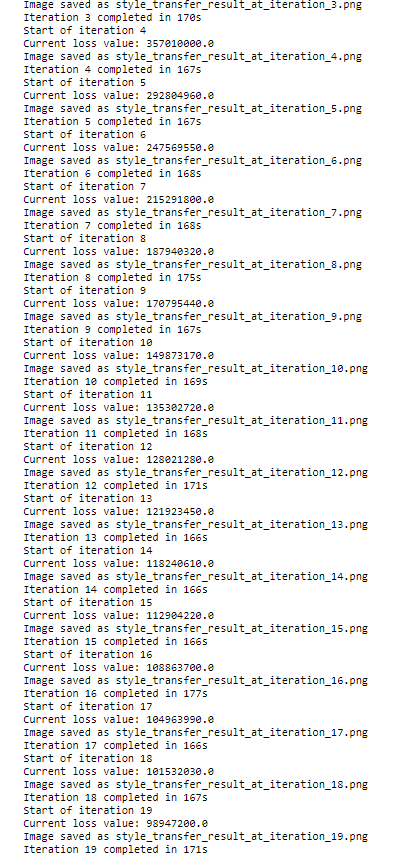## 8.4 用变分自编码器生成图像

### 8.4.1 从图像的潜在空间采样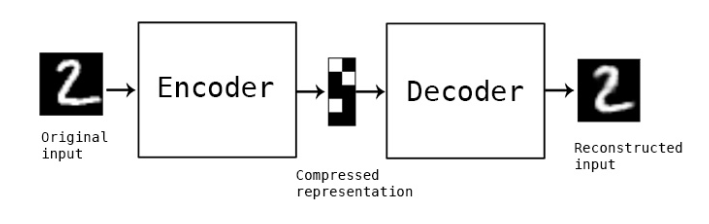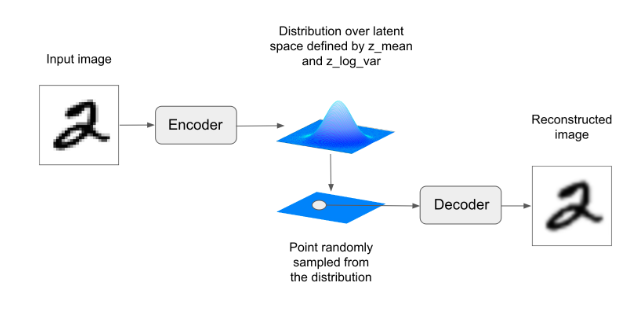### 8.4.3 变分自编码器

# Encode the input into a mean and variance parameter
z_mean, z_log_variance = encoder(input_img)

# Draw a latent point using a small random epsilon
z = z_mean + exp(z_log_variance) * epsilon

# Then decode z back to an image
reconstructed_img = decoder(z)

# Instantiate a model
model = Model(input_img, reconstructed_img)

# Then train the model using 2 losses:
# a reconstruction loss and a regularization loss


# 8-23 VAE编码器网络
import keras
from keras import layers
from keras import backend as K
from keras.models import Model
import numpy as np

img_shape = (28, 28, 1)
batch_size = 16
latent_dim = 2  # Dimensionality of the latent space: a plane

input_img = keras.Input(shape=img_shape)

x = layers.Conv2D(32, 3,
x = layers.Conv2D(64, 3,
strides=(2, 2))(x)
x = layers.Conv2D(64, 3,
x = layers.Conv2D(64, 3,
shape_before_flattening = K.int_shape(x)

x = layers.Flatten()(x)
x = layers.Dense(32, activation='relu')(x)

z_mean = layers.Dense(latent_dim)(x)
z_log_var = layers.Dense(latent_dim)(x)


# 8-24 潜在空间采样的函数
def sampling(args):
z_mean, z_log_var = args
epsilon = K.random_normal(shape=(K.shape(z_mean), latent_dim),
mean=0., stddev=1.)
return z_mean + K.exp(z_log_var) * epsilon

z = layers.Lambda(sampling)([z_mean, z_log_var])

# 8-25 VAE解码器网络，将潜在空间点映射为图像
# This is the input where we will feed z.
decoder_input = layers.Input(K.int_shape(z)[1:])

# Upsample to the correct number of units
x = layers.Dense(np.prod(shape_before_flattening[1:]),
activation='relu')(decoder_input)

# Reshape into an image of the same shape as before our last Flatten layer
x = layers.Reshape(shape_before_flattening[1:])(x)

# We then apply then reverse operation to the initial
# stack of convolution layers: a Conv2DTranspose layers
# with corresponding parameters.
x = layers.Conv2DTranspose(32, 3,
strides=(2, 2))(x)
x = layers.Conv2D(1, 3,
# We end up with a feature map of the same size as the original input.

# This is our decoder model.
decoder = Model(decoder_input, x)

# We then apply it to z to recover the decoded z.
z_decoded = decoder(z)


# 8=26 用于计算VAE损失的自定义层
class CustomVariationalLayer(keras.layers.Layer):

def vae_loss(self, x, z_decoded):
x = K.flatten(x)
z_decoded = K.flatten(z_decoded)
xent_loss = keras.metrics.binary_crossentropy(x, z_decoded)
kl_loss = -5e-4 * K.mean(
1 + z_log_var - K.square(z_mean) - K.exp(z_log_var), axis=-1)
return K.mean(xent_loss + kl_loss)

def call(self, inputs):
x = inputs
z_decoded = inputs
loss = self.vae_loss(x, z_decoded)
# We don't use this output.
return x

# We call our custom layer on the input and the decoded output,
# to obtain the final model output.
y = CustomVariationalLayer()([input_img, z_decoded])


# 8-27 训练VAE
from keras.datasets import mnist

vae = Model(input_img, y)
vae.compile(optimizer='rmsprop', loss=None)
vae.summary()

# Train the VAE on MNIST digits
(x_train, _), (x_test, y_test) = mnist.load_data()

x_train = x_train.astype('float32') / 255.
x_train = x_train.reshape(x_train.shape + (1,))
x_test = x_test.astype('float32') / 255.
x_test = x_test.reshape(x_test.shape + (1,))

vae.fit(x=x_train, y=None,
shuffle=True,
epochs=10,
batch_size=batch_size,
validation_data=(x_test, None))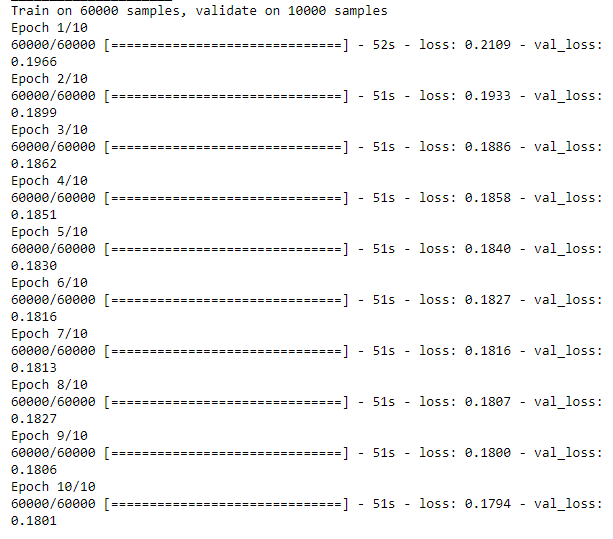# 8-28 从二维潜在空间中采样一组点的网络，并解码为图像
import matplotlib.pyplot as plt
from scipy.stats import norm

# Display a 2D manifold of the digits
n = 15  # figure with 15x15 digits
digit_size = 28
figure = np.zeros((digit_size * n, digit_size * n))
# Linearly spaced coordinates on the unit square were transformed
# through the inverse CDF (ppf) of the Gaussian
# to produce values of the latent variables z,
# since the prior of the latent space is Gaussian
grid_x = norm.ppf(np.linspace(0.05, 0.95, n))
grid_y = norm.ppf(np.linspace(0.05, 0.95, n))

for i, yi in enumerate(grid_x):
for j, xi in enumerate(grid_y):
z_sample = np.array([[xi, yi]])
z_sample = np.tile(z_sample, batch_size).reshape(batch_size, 2)
x_decoded = decoder.predict(z_sample, batch_size=batch_size)
digit = x_decoded.reshape(digit_size, digit_size)
figure[i * digit_size: (i + 1) * digit_size,
j * digit_size: (j + 1) * digit_size] = digit

plt.figure(figsize=(10, 10))
plt.imshow(figure, cmap='Greys_r')
plt.show()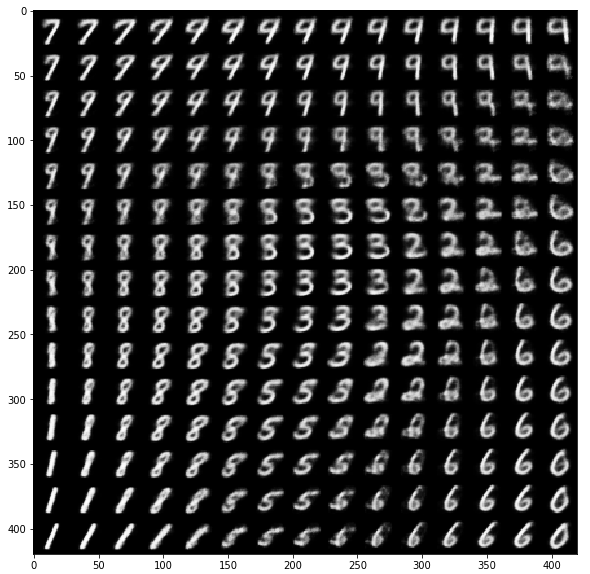## 8.5 生成式对抗网络

### 8.5.1 GAN的简要实现流程

1. generator网络将行政为（latent_dim，）的向量映射到形状为（32，32，3）的图像。
2. discriminator网络将形状为（32，32，3）的图像映射到一个二进制分数，用于评估图像为真的概率。
3. gan网络将g网络和d网络连接在一起：gan(x) = d(g(x))
4. 使用真、假标签的真假图像样本来训练判别器
5. 训练生成器，使用gan模型的损失相对于生成器权重的梯度。训练生成器来欺骗判别器。

### 8.5.2 大量技巧

1. 使用tanh作为生成器最后一层的激活，而不用sigmoid
2. 使用正太分布对潜在空间的点进行采样，而不用均匀分布
3. 随机性能提高稳健性。训练GAN得到一个动态平衡。在判别器中使用dropout，想判别器的标签加入随机噪声。
4. 稀疏的梯度会妨碍训练。使用LeakyReLu代替ReLU激活
5. 使用Conv2DTranspose，内核大小要被步幅大小整除

### 8.5.3 生成器

# 8-29 GAN生成器网络
import keras
from keras import layers
import numpy as np

latent_dim = 32
height = 32
width = 32
channels = 3

generator_input = keras.Input(shape=(latent_dim,))

# First, transform the input into a 16x16 128-channels feature map
x = layers.Dense(128 * 16 * 16)(generator_input)
x = layers.LeakyReLU()(x)
x = layers.Reshape((16, 16, 128))(x)

# Then, add a convolution layer
x = layers.LeakyReLU()(x)

# Upsample to 32x32
x = layers.Conv2DTranspose(256, 4, strides=2, padding='same')(x)
x = layers.LeakyReLU()(x)

# Few more conv layers
x = layers.LeakyReLU()(x)
x = layers.LeakyReLU()(x)

# Produce a 32x32 1-channel feature map
x = layers.Conv2D(channels, 7, activation='tanh', padding='same')(x)
generator = keras.models.Model(generator_input, x)
generator.summary()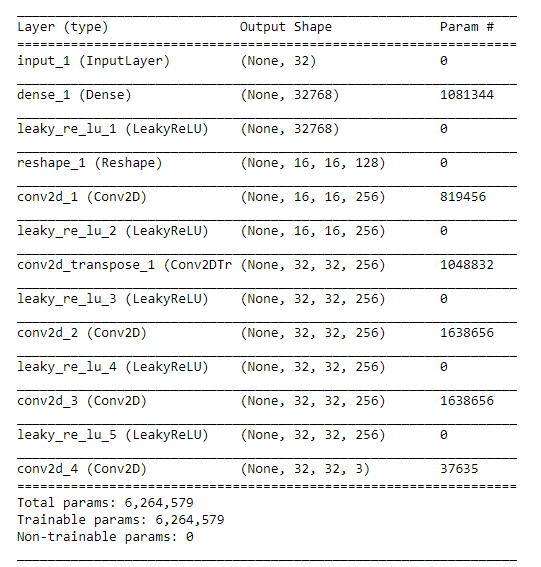### 8.5.4 判别器

# 8-30 GAN判别器网络
discriminator_input = layers.Input(shape=(height, width, channels))
x = layers.Conv2D(128, 3)(discriminator_input)
x = layers.LeakyReLU()(x)
x = layers.Conv2D(128, 4, strides=2)(x)
x = layers.LeakyReLU()(x)
x = layers.Conv2D(128, 4, strides=2)(x)
x = layers.LeakyReLU()(x)
x = layers.Conv2D(128, 4, strides=2)(x)
x = layers.LeakyReLU()(x)
x = layers.Flatten()(x)

# One dropout layer - important trick!
x = layers.Dropout(0.4)(x)

# Classification layer
x = layers.Dense(1, activation='sigmoid')(x)

discriminator = keras.models.Model(discriminator_input, x)
discriminator.summary()

# To stabilize training, we use learning rate decay
# and gradient clipping (by value) in the optimizer.
discriminator_optimizer = keras.optimizers.RMSprop(lr=0.0008, clipvalue=1.0, decay=1e-8)
discriminator.compile(optimizer=discriminator_optimizer, loss='binary_crossentropy')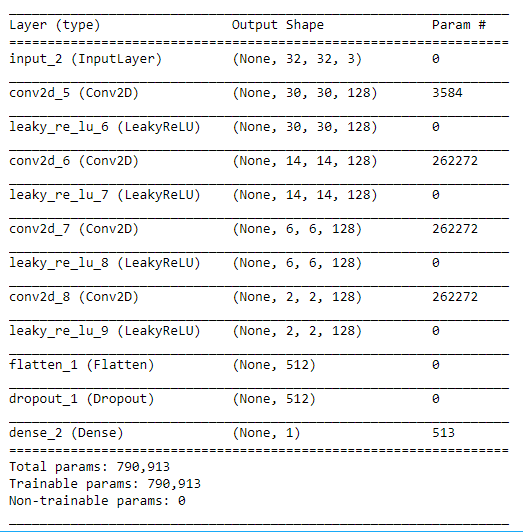### 8.5.5 对抗网络

# 8-31 对抗网络
# Set discriminator weights to non-trainable
# (will only apply to the gan model)
discriminator.trainable = False

gan_input = keras.Input(shape=(latent_dim,))
gan_output = discriminator(generator(gan_input))
gan = keras.models.Model(gan_input, gan_output)

gan_optimizer = keras.optimizers.RMSprop(lr=0.0004, clipvalue=1.0, decay=1e-8)
gan.compile(optimizer=gan_optimizer, loss='binary_crossentropy')


### 8.5.6 如何训练DCGAN

# 8-32 实现GAN的训练
import os
from keras.preprocessing import image

(x_train, y_train), (_, _) = keras.datasets.cifar10.load_data()

# Select frog images (class 6)
x_train = x_train[y_train.flatten() == 6]

# Normalize data
x_train = x_train.reshape(
(x_train.shape,) + (height, width, channels)).astype('float32') / 255.

iterations = 10000
batch_size = 20
save_dir = '/home/ubuntu/gan_images/'

# Start training loop
start = 0
for step in range(iterations):
# Sample random points in the latent space
random_latent_vectors = np.random.normal(size=(batch_size, latent_dim))

# Decode them to fake images
generated_images = generator.predict(random_latent_vectors)

# Combine them with real images
stop = start + batch_size
real_images = x_train[start: stop]
combined_images = np.concatenate([generated_images, real_images])

# Assemble labels discriminating real from fake images
labels = np.concatenate([np.ones((batch_size, 1)),
np.zeros((batch_size, 1))])
# Add random noise to the labels - important trick!
labels += 0.05 * np.random.random(labels.shape)

# Train the discriminator
d_loss = discriminator.train_on_batch(combined_images, labels)

# sample random points in the latent space
random_latent_vectors = np.random.normal(size=(batch_size, latent_dim))

# Assemble labels that say "all real images"

# Train the generator (via the gan model,
# where the discriminator weights are frozen)

start += batch_size
if start > len(x_train) - batch_size:
start = 0

# Occasionally save / plot
if step % 100 == 0:
# Save model weights
gan.save_weights('gan.h5')

# Print metrics
print('discriminator loss at step %s: %s' % (step, d_loss))
print('adversarial loss at step %s: %s' % (step, a_loss))

# Save one generated image
img = image.array_to_img(generated_images * 255., scale=False)
img.save(os.path.join(save_dir, 'generated_frog' + str(step) + '.png'))

# Save one real image, for comparison
img = image.array_to_img(real_images * 255., scale=False)
img.save(os.path.join(save_dir, 'real_frog' + str(step) + '.png'))

import matplotlib.pyplot as plt

# Sample random points in the latent space
random_latent_vectors = np.random.normal(size=(10, latent_dim))

# Decode them to fake images
generated_images = generator.predict(random_latent_vectors)

for i in range(generated_images.shape):
img = image.array_to_img(generated_images[i] * 255., scale=False)
plt.figure()
plt.imshow(img)

plt.show()


2019-07-12 16:53:40 wangwei19871103 阅读数 303
• ###### 深度学习入门及如何转型AI领域

深度学习入门转型视频教程，该课程主要告诉开发者如何快速入门深度学习。讲师是在机器学习，深度学习神经网络领域多年开发研究经验，精通算法原理与编程实践。曾完成过多项图像识别，目标识别，语音识别的实际项目，经验丰富。关注深度学习领域各种开源项目，如TensorFlow，Caffe，Torch等。喜欢理论与实践相结合的教学风格，课程编排由浅入深，体系清晰完整。

2772 人正在学习 去看看 CSDN讲师

# 序列生成器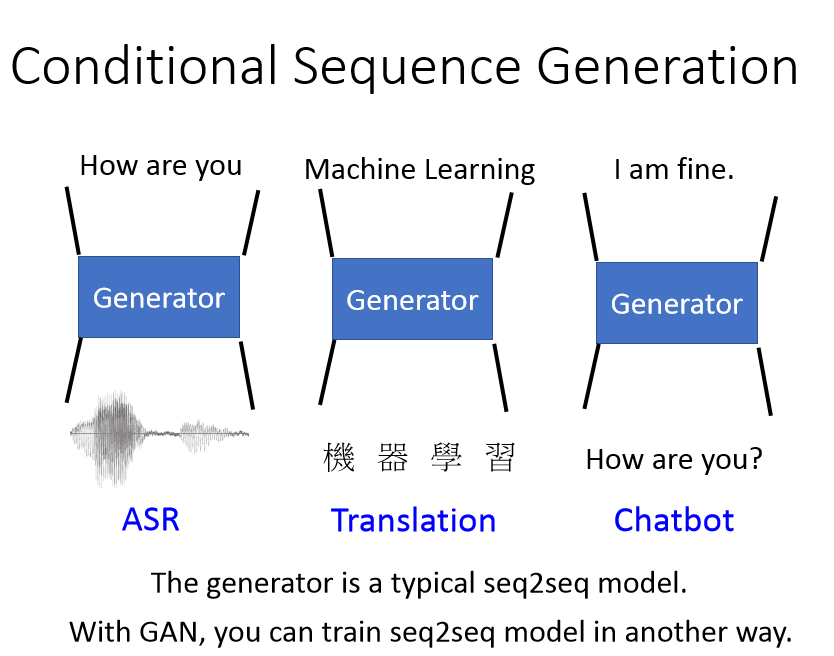## 机器人聊天问题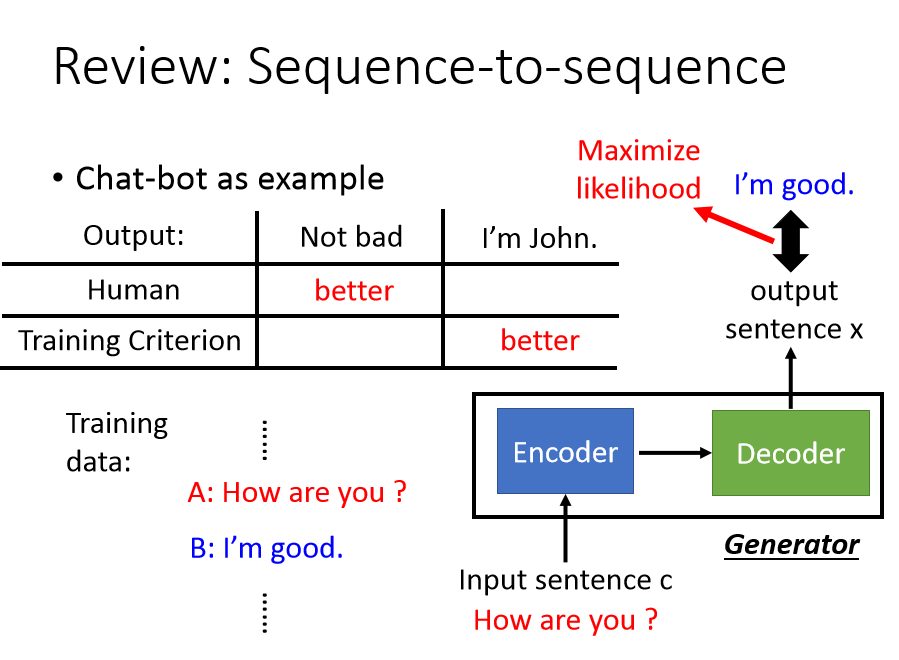## 改进序列模型